## How to Concatenate Text based on unique values in Another Column in Excel

This post will guide you how to concatenate text values based on unique values in another column in Excel. How do I concatenate cells based on specific criteria in Excel.

## 1. Concatenate Text Based on unique Values in Another Column

Assuming that you have a list of data in range A1:B6, in which contain product IDs and product Names. And you want to concatenate product names based on unique ID values (There are duplicated ID values in Column A), How to do it. You need to extract unique product IDs in another range, and then concatenating text values based on newly created range with a User Defined Function. Here are the steps:

Step1: you can use an Excel Array formula based on the IFERROR function, the INDEX function, the MATCH function and the COUNTIF function to extract the unique product ID values.

``=IFERROR(INDEX(\$A\$2:\$A\$6, MATCH(0,COUNTIF(\$C\$1:C1, \$A\$2:\$A\$6), 0)),"")``

Type this formula into cell C2, and press Ctrl + Shift + Enter keys on your keyboard to change it as array formula.  And then drag the AutoFill Handle down to other cells until getting blank cells.

Step2: open your excel workbook and then click on “Visual Basic” command under DEVELOPER Tab, or just press “ALT+F11” shortcut.

Step3: then the “Visual Basic Editor” window will appear.

Step4: click “Insert” ->”Module” to create a new module.

Step5: paste the below VBA code (code from here) into the code window. Then clicking “Save” button.

```Function Combinerows(CriteriaRng As Range, Criteria As Variant, _
ConcatenateRng As Range, Optional Delimeter As String = " , ") As Variant
Dim i As Long
Dim strResult As String
On Error GoTo ErrHandler
If CriteriaRng.Count <> ConcatenateRng.Count Then
Combinerows = CVErr(xlErrRef)
Exit Function
End If

For i = 1 To CriteriaRng.Count
If CriteriaRng.Cells(i).Value = Criteria Then
strResult = strResult & Delimeter & ConcatenateRng.Cells(i).Value
End If
Next i

If strResult <> "" Then
strResult = Mid(strResult, Len(Delimeter) + 1)
End If

Combinerows = strResult
Exit Function
ErrHandler:
Combinerows = CVErr(xlErrValue)
End Function```

Step6: back to the current worksheet, then type the following formula in a blank cell, and then press Enter key.

``=combinerows(A2:A6,C2,B2:B6)``

Step7: drag the AutoFill handle over other cells to  concatenate text based on unique product ID values.

## 2. Video: Concatenate Text based on unique values in Another Column

This video will show how to use a formula in combination with a User defined function with VBA code to concatenate text based on unique values in another column in Excel.

## 3. Related Functions

• Excel IFERROR function
The Excel IFERROR function returns an alternate value you specify if a formula results in an error, or returns the result of the formula.The syntax of the IFERROR function is as below:= IFERROR (value, value_if_error)…
• Excel COUNTIF function
The Excel COUNTIF function will count the number of cells in a range that meet a given criteria. This function can be used to count the different kinds of cells with number, date, text values, blank, non-blanks, or containing specific characters.etc.= COUNTIF (range, criteria)…
• Excel INDEX function
The Excel INDEX function returns a value from a table based on the index (row number and column number)The INDEX function is a build-in function in Microsoft Excel and it is categorized as a Lookup and Reference Function.The syntax of the INDEX function is as below:= INDEX (array, row_num,[column_num])…
• Excel MATCH function
The Excel MATCH function search a value in an array and returns the position of that item.The syntax of the MATCH function is as below:= MATCH  (lookup_value, lookup_array, [match_type])….

## VLOOKUP Returns zero instead of #NA in Excel

This post will guide you how to VLookup and return zero instead of #N/A in Excel. How do I use VLookup function and return zero instead of #N/A if not found in Excel. How to display zero instead of #N/A when using VLOOKUP in Excel. How to replace #N/A with Zero when VLOOKUP.

## 1. VLOOKUP Returns zero instead of #N/A

The VLOOKUP function is one of the most useful function to find data in a given range of cells in Excel. And if the VLOOKUP function cannot find the result that it is looking for, and it will display a #N/A error. And if you would like it to display a “0” instead of #N/A. How to achieve it.

Assuming that you have a list of data in range B1:C7 which contain the product names and sales data, and you want to use Vlookup function to lookup the product “outlook” in range B1:C7, and the return the sales value in sales column.

Type the following formula in a blank cell and then press Enter key in your keyboard.

``=IFERROR(VLOOKUP("outlook",\$B\$1:\$C\$7,2,0),0)``

From the returned result, you can know that the error message #N/A has been replaced with number 0.

Let’s try to look the product “excel ” in range B1:C7, type the following formula in a blank cell:

``=IFERROR(VLOOKUP("excel",\$B\$1:\$C\$7,2,0),0)``

The sales value for product excel has been extracted from the sales column.

## 2. Video:VLOOKUP Returns zero instead of #N/A

This video will show you how to modify the VLOOKUP formula to return zero instead of #NA in Excel.

## 3. Related Functions

• Excel VLOOKUP function
The Excel VLOOKUP function lookup a value in the first column of the table and return the value in the same row based on index_num position.The syntax of the VLOOKUP function is as below:= VLOOKUP (lookup_value, table_array, column_index_num,[range_lookup])….
• Excel IFERROR function
The Excel IFERROR function returns an alternate value you specify if a formula results in an error, or returns the result of the formula.The syntax of the IFERROR function is as below:= IFERROR (value, value_if_error)….

## How to remove non numeric characters from a cell

This post explains that how to remove non-numeric characters (numbers) from a text string in one cell in excel 2016/2019/365. How to remove non numeric characters from a cell containing text string with an excel formula. And how to remove alphanumeric characters from a cell with a user defined function in excel VBA.

## 1. Remove non numeric characters with an Excel Formula

If you want to remove non numeric characters from a text cell in excel, you can use the array formula:

``{=TEXTJOIN("",TRUE,IFERROR(MID(B1,ROW(INDIRECT("1:"&LEN(B1))),1)+0,""))}``

Let’s see how the above formula works:

=ROW(INDIRECT(“1:”&LEN(B1))

The ROW function returns the below array list:

``{1,2,3,4,5,6,7,8,9}``

=MID(A1,ROW(INDIRECT(“1:”&LEN(A1))),1)

The MID formula will return the below array:

``{"e","x","c","e","l","2","0","1","6"}``

=IFERROR(MID(B1,ROW(INDIRECT(“1:”&LEN(B1))),1)+0,””)

The array returned by the above MID function add zero for each value in array. If the value is a numeric text, it will be converted to text format. If not, returns empty string. So the IFERROR function returns the below array:

``{2,0,1,6}``

Last, the TEXTJOIN function join the values in above array returned by the IFERROR function.

## 2. Remove non numeric characters using VBA Code

You can create a new function to remove numeric characters from a cell that contain text string in Excel VBA. Just refer to the below steps:

Step1: open visual Basic Editor, then insert a module and name as : RemoveNonNum.

Step2: click “Insert“->”Module“, then paste the following VBA code into the window:

Step3: paste the below VBA code into the code window. Then clicking “Save” button.

```Sub RemoveNonNum()
Set myRange = Application.Selection
Set myRange = Application.InputBox("select one Range that you want to remove non numeric characters", "RemoveNonNum", myRange.Address, Type:=8)
For Each myCell In myRange
LastString = ""
For i = 1 To Len(myCell.Value)
mT = Mid(myCell.Value, i, 1)
If mT Like "[0-9]" Then
tString = mT
Else
tString = ""
End If
LastString = LastString & tString
Next i
myCell.Value = LastString
Next
End Sub```

Step4: back to the current worksheet, then run the above excel macro. Click Run button.

Step5: select one Range that you want to remove non numeric characters. click Ok button.

Step6: Let’s see the last result:

## 3. Video: Remove non numeric characters in Excel

This video will demonstrate how to remove non-numeric characters in Excel using a formula or VBA code.

## 4. Related Formulas

• Remove Numeric Characters from a Cell
If you want to remove numeric characters from alphanumeric string, you can use the following complex array formula using a combination of the TEXTJOIN function, the MID function, the Row function, and the INDIRECT function..…
• Combine Text from Two or More Cells into One Cell
If you want to combine text from multiple cells into one cell and you can use the Ampersand (&) symbol.If you are using the excel 2016, then you can use a new function TEXTJOIN function to combine text from multiple cells…

## 5. Related Functions

• Excel TEXTJOIN function
The Excel TEXTJOIN function joins two or more text strings together and separated by a delimiter. you can select an entire range of cell references to be combined in excel 2016.The syntax of the TEXTJOIN function is as below:= TEXTJOIN  (delimiter, ignore_empty,text1,[text2])…
• Excel MID function
The Excel MID function returns a substring from a text string at the position that you specify.The syntax of the MID function is as below:= MID (text, start_num, num_chars)…
• Excel LEN function
The Excel LEN function returns the length of a text string (the number of characters in a text string).The LEN function is a build-in function in Microsoft Excel and it is categorized as a Text Function.The syntax of the LEN function is as below:= LEN(text)…
• Excel IFERROR function
The Excel IFERROR function returns an alternate value you specify if a formula results in an error, or returns the result of the formula.The syntax of the IFERROR function is as below:= IFERROR (value, value_if_error)….
• Excel ROW function
The Excel ROW function returns the row number of a cell reference.The ROW function is a build-in function in Microsoft Excel and it is categorized as a Lookup and Reference Function.The syntax of the ROW function is as below:= ROW ([reference])….
• Excel INDIRECT  function
The Excel ROW function returns the row number of a cell reference.The ROW function is a build-in function in Microsoft Excel and it is categorized as a Lookup and Reference Function.The syntax of the ROW function is as below:= ROW ([reference])….

## How to Find the Position of First Number in A Text String in Excel

This post will guide you how to get the position of the first number in a text string in Excel. How do I find the position of first number in a text string in Excel 2013/2016.

## 1. Find the Position of First Number in a Cell using Formula

Assuming that you have a list of data in range B1:B4, in which contain text strings. And you wish to get the position of the first number in those range of cells. How to accomplish it. And you can use an formula based on the MIN function and the SEARCH function. Like below:

``=MIN(SEARCH({0,1,2,3,4,5,6,7,8,9},B1&"0123456789"))``

Type this formula into a blank cell and press Enter key on your keyboard, and drag the AutoFill Handle down to other cells to apply this formula.

You would see that the position of the first number is calculated.

If you want to get the position of last number in a text string in your range of cells, you can use another array formula based on the MAX function, the IFERROR function, the FIND function, the ROW function, and the LEN function. Like this:

``=MAX(IFERROR(FIND({1,2,3,4,5,6,7,8,9,0},B1,ROW(INDIRECT("1:"&LEN(B1)))),0))``

Type this formula into a blank cell and press Ctrl + Shift + Enter keys on your keyboard, and drag the AutoFill Handle down to other cells to apply this formula.

## 2. Find the Position of First Number in a Cell using User Defined Function with VBA Code

You can also create a User defined function with VBA Code to find the position of first number in a given cell in Excel. here are the steps:

Step1: press Alt + F11 to open the Visual Basic Editor.

Step2: In the Visual Basic Editor, click Insert > Module to insert a new module.

Step3: Copy the below VBA code for the custom function and paste it into the new module. Save the VBA module by clicking File > Save or by pressing Ctrl + S.

```Function FirstNumberPosition_Excelhow(rng As Range) As Integer

Dim s As String
Dim i As Integer

s = rng.Value

For i = 1 To Len(s)
If IsNumeric(Mid(s, i, 1)) Then
FirstNumberPosition_Excelhow = i
Exit Function
End If
Next i

End Function```

Step4: Type the following formula in a blank cell:

``=FirstNumberPosition_Excelhow(B1)``

Step5: Press Enter to calculate the result of the formula using the custom function.

## 3. Video: Find the Position of First Number in a Cell

This video will demonstrate how to find the position of the first number in a cell using a formula or VBA code in Excel.

## How to Get Text before or after Dash Character in Excel

This post will guide you how to get text before or after dash character in a given cell in Excel. How do I extract text string before or after dash character in Excel.

## 1. Video: Get Text before or after Dash Character in Excel

You can watch this short video tutorial to learn how to easily extract text before or after a dash character in Excel using simple functions.

## 2. Get Text before Dash Character with Formula

Assuming that you have a list of data in range B1:B5, which contain text string. And you want to extract text before or after dash character in a given cell or range. How to do it. You can use a formula based on the LEFT function and the FIND function to extract text before dash character in a given cell in Excel. Like this:

``=LEFT(B2,FIND("-",B2)-1)``

Select the cell where you want to display the result, then type this formula into a blank cell and press Enter key on your keyboard, and drag the AutoFill handle down to other cells to apply this formula to extract text before dash.

You would notice that all text values before dash character have been extracted into new cells.

## 3. Get Text after Dash Character with Formula

If you want to get text values after dash character from a given cell or range in Excel, you can use another formula based on the REPLACE function and the Find function. Like this:

``=REPLACE(B2,1,FIND("-",B2),"")``

Type this formula into a blank cell and press Enter key on your keyboard, and drag the AutoFill handle down to other cells to apply this formula to extract text after dash.

Let’s see how this formula works:

The Find function will return the position of the first dash character from text string in Cell B1, and pass the returned value to REPLACE function as its second argument.

The REPLACE function will replace all text string from the first character to the first dash character. So that you can get text string after dash character.

To avoid this problem, you can use the IFERROR function in combination with the above formula to display a blank cell instead.

``=IFERROR(REPLACE(B2,1,FIND("-",B2),""),"")``

## 4. Get Text Values before and after Dash Character with Text to Column

You can also use Text to Column feature to achieve the same result of extracting text string before and after dash character from the selected range of cells in Excel. Here are the steps:

Step1: select your data that you want to split text string by dash.

Step2: go to DATA tab, click Text to Columns command under Data Tools group. And the Convert Text to columns Wizard dialog will open.

Step3: choose Delimited radio button under Original data type section. Click OK button.

Step4: only check Other check box under Delimiters section, and enter dash (-) character into the Other text box, and click Next button.

Step5: select one Destination cell to place text strings or just click Finish button.

You would notice that the selected cells have been split into two columns by dash character. And one column contain the text string before dash from the original text string. And another column contain the text string after dash character.

## 5. Related Functions

• Excel Replace function
The Excel DATE function returns the serial number for a date.The syntax of the DATE function is as below:= DATE (year, month, day) …
• Excel LEFT function
The Excel LEFT function returns a substring (a specified number of the characters) from a text string, starting from the leftmost character.The LEFT function is a build-in function in Microsoft Excel and it is categorized as a Text Function.The syntax of the LEFT function is as below:= LEFT(text,[num_chars])…t)…
• Excel FIND function
The Excel FIND function returns the position of the first text string (sub string) within another text string.The syntax of the FIND function is as below:= FIND(find_text, within_text,[start_num])…

## Trap Error or Replace Error by Specific Value with IFERROR function

We often use Excel formulas in our work life, and we may encounter this situation that the formula throws an error and finally an error like #DIV/0! Is displayed in the cell. In today’s tutorial, we will introduce you the application of Excel IFERROR function to catch errors and replace errors by specific values per your requirement.

In today’s example, if the IFERROR formula returns a normal value, we keep the normal value, and if it throws an error, we will return a specific value or a text to mark the error type.

Using a simple division as an example, the division reports error #DIV/0! if the divisor is zero. We use the following example to show you how to use Excel IFERROR function to return a specific value in Excel worksheet if #DIV/0! error is detected.

In this example, if the cell in Piece Count column is not empty, the formula will return the unit price calculated by Total/Piece; And if the cell in Piece Count column is empty, the formula will return the value saved in Total column instead.

Notes:

In order to get the return value with \$, we need to set the cell format to “Currency” before entering the formula. You can set “Currency” via Home->Number->Number Format dropdown list.

## FORMULA

In this example, we have used only the IFERROR function without nesting other functions.

Excel IFERROR function detects if a formula or expression is in error and returns a specific value if the formula evaluates to an error. It returns the result of the formula or expression if there is no error.

Syntax:

`=IFERROR(value, value_if_error) `

In this example, we can directly apply this function with entering our own value and value if error.

`= IFERROR(B2/C2, B2)`

## EXPLANATION

Normally, if we just enter =B2/C2 without applying Excel IFERROR function in unit price column, #DIV/0! error will be displayed if piece count is empty.

After introducing Excel IFERROR function, we can know that if error is thrown by the input expression or formula, we can record the error by setting a specific value (argument “value_if_error”) in IFERROR, then the normal error will be replaced by this value. This value can be a cell reference, a fixed value, a formula, or an error mark like “error code1”.

### RETURN A SPECIFIC VALUE

In this example, we want to get the unit price by Total/Piece Count. We notice that in Piece Count column, some cells are empty, which means that the divisor is empty. In this case, we want to keep their total value as their unit price, so if we encounter an error like this, the total value will be returned by IFERROR.

Calculate B2/C2 in the formula bar:

`=IFERROR(333.333333333333,1000)`

Expression B2/C2 returns value 333.3333 and doesn’t throw an error, so the result of the expression is displayed in D2.

Calculate B4/C4 in the formula bar:

`=IFERROR(#DIV/0!,3000)`

This time expression B4/C4 returns an error #DIV/0!, IFERROR detects this error and comes to value_if_error, we set value_if_error to B4, so value 3000 in B4 is returned by this formula.

### RETURN A TEXT

We can replace error value with some other values. For example, we can enter text “ERROR” in the formula, if error is detected by Excel IFERROR function, “ERROR” is recorded in unit price column.

The formula is:

`=IFERROR(B2/C2,"ERROR")`

Notes:

If text is entered in a formula, the entered text must be enclosed in double quotation marks. Otherwise, an error #NAME? will be thrown.

### Related Functions

• Excel IFERROR function
The Excel IFERROR function returns an alternate value you specify if a formula results in an error, or returns the result of the formula.The syntax of the IFERROR function is as below:= IFERROR (value, value_if_error)….

## Find and Replace Multiple Values

This post will guide you how to find and replace multiple values at once with VBA macro or using formula  in Excel. How do I make multiple find and replace in Excel.

Suppose that you have a few cells containing few values and you want to find and replace two or three values; then you might think that it’s not a big deal; because you would prefer to do it with the help of the Find and Replace built-in feature of excel, then congratulation because you have supposed to choose the right way for this task.

There isn’t any doubt that you can find and replace values in excel by its` Find and Replace` built-in feature, which works entirely perfect for two or three values, but when you would have the bulk of values, and you want to find and replace them, then doing this task manually by the aid of this `Find and Replace `built-in feature would be a stupid decision because you would get tired of it and would never complete your work on time.

But there isn’t any need to worry about it because, luckily, there are a few effective methods for finding and replacing multiple values in a few seconds.

After reading this article carefully, you would get to know the several ways to find and replace multiple words, individual characters, or strings so that you can select the best one according to your needs and ease.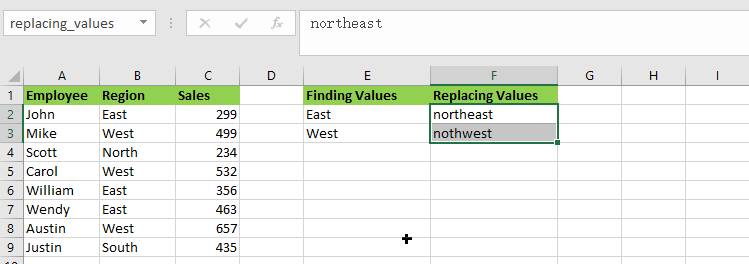So let’s get straight into it!

## Find and Replay Multiple Values using Formula

### General formula:

The formula given below would help you out for finding and replacing multiple values within few seconds:

`=SUBSTITUTE(SUBSTITUTE(B2,INDEX(finding_values,1),INDEX(replacing_values,1)),INDEX(finding_values,2),INDEX(replacing_values,2))`

or

`=SUBSTITUTE(SUBSTITUTE(B2,finding_values,replacing_values), finding_values, replacing_values))`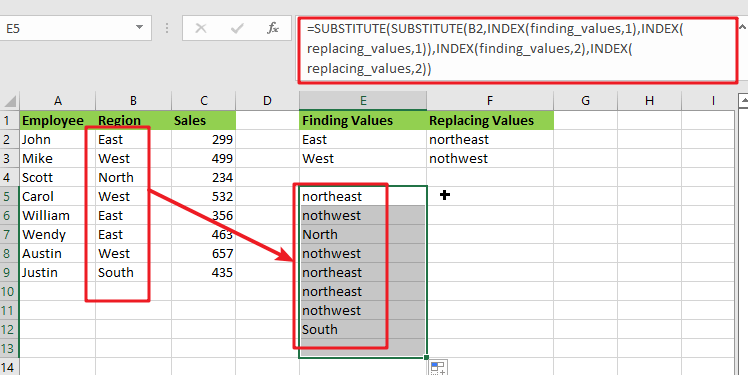### Syntax Explanation:

Before we dive into the formula for getting the job done effectively, we need to understand each syntax so that we can know how each syntax helps to find and replace the multiple values:

• `SUBSTITUTE`: This function lets users replace current text in a text string with new text when they desire to replace text based on its content rather than its position.
• `INDEX`: The INDEX function in Excel is used to repeat characters a specified number of times.
• `B``2`: This is the input value.
• `Find`: This command specifies the text to be found in the input range.
• `Replace`: It represents the text that is being replaced.
• `The comma sign` (,): is a separator that may separate a list of values.
• `Parenthesis` (): The primary purpose of the parenthesis () symbol is to organize the components.

### Let’s See How This Formula Works:

As I said above, there are a few ways to find and replace multiple values in Excel in a matter of seconds, and one of the most popular and simple methods is to utilize the nested SUBSTITUTE function.

The logic of this formula is very simple: you write a few individual functions to replace an old value with a new one. Then you nest those functions one inside the other so that each subsequent SUBSTITUTE uses the output of the previous SUBSTITUTE to find the next value.

`=SUBSTITUTE(SUBSTITUTE(Text_values,finding_values,replacing_values), finding_values, replacing_values))`

Assume you wish to replace the region names in the `B2:B9` list of places with the replacing names.

For getting the desired output, input the old values in` E2:E3` and the new values in `F2:F3`, as seen in the image and formula below. Then, in `E5`, enter the following formula:

`= SUBSTITUTE(SUBSTITUTE(B2, E2, F2), E3,F3)`

You’ll have completed all of the replacements at once:

Please remember that the above method only works in Excel 365, which supports dynamic arrays.

In pre-dynamic versions of Excel 2016, Excel 2019, and prior,type the following formula:

`= SUBSTITUTE(SUBSTITUTE(B2, \$E\$2, \$F\$2), \$E\$3,\$F\$3)`

Also, note that in this case, we lock the replacement values with absolute cell references to ensure that they do not shift when copying the formula down.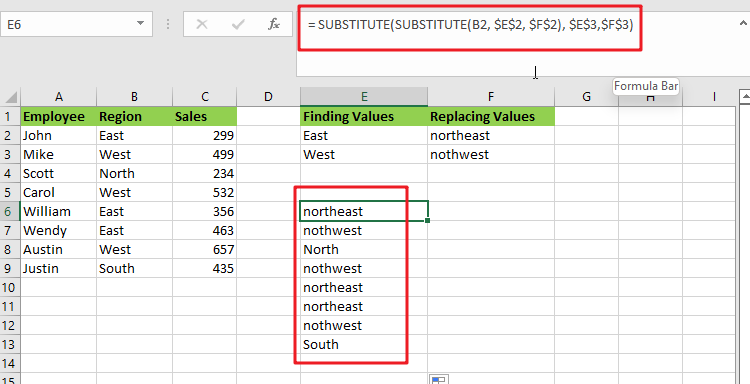The SUBSTITUTE function is case-sensitive, so you must type the old values (old text) in the same letter case as the original data.

This method has a significant drawback: It should only be used for a limited number of find/replace values. This nested function becomes extremely difficult to manage when you have dozens of values to replace.

Benefits: Simple to deploy; compatible with all Excel versions

### Find and replace multiple values using XLOOKUP Function

The XLOOKUP function is very efficient and helpful while replacing the entire cell content rather than just a portion of it.

Assume you have a list of region in column B and want to replace all the “East” or “West” regions as “northeast” or “northwest” values. Enter the following formula in B2:

`=XLOOKUP(B2,\$E\$2:\$E\$3,\$F\$2:\$F\$3,B2)`

The formula does the following when translated from Excel to human language:

It searches the B2 value (lookup value) in E2:E3 (lookup array) and returns a match from F2:F3 (return array). If the original value cannot be retrieved, take it from B2.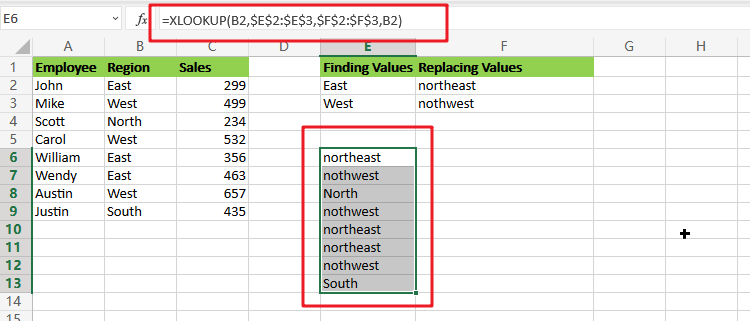Because the XLOOKUP function is only accessible in Excel 365. However, you can easily replicate this behavior using a combination of IFERROR or IFNA and VLOOKUP:

`=IFNA(VLOOKUP(B2,\$E\$2:\$F\$3,2,FALSE),B2)`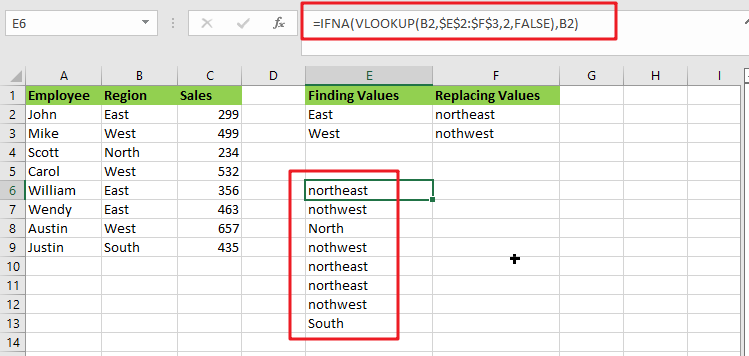Unlike the SUBSTITUTE, the XLOOKUP and VLOOKUP functions are both not case-sensitive, which means they search for lookup values regardless of letter case.

## Find And Replace Multiple Values with VBA Macro

Assuming that you have a list of data in range B1:B6, and you want to find multiple values and replace those value with different values. For example, find “excel” string and replace its as excel2013, and find “word’’ string and replace its as word2013, and so on. How to achieve it. You should use an excel VBA macro to quickly find and replace multiple values. Just do the following steps:

#1 open your excel workbook and then click on “Visual Basic” command under DEVELOPER Tab, or just press “ALT+F11” shortcut.#2 then the “Visual Basic Editor” window will appear.

#3 click “Insert” ->”Module” to create a new module.#4 paste the below VBA code into the code window. Then clicking “Save” button.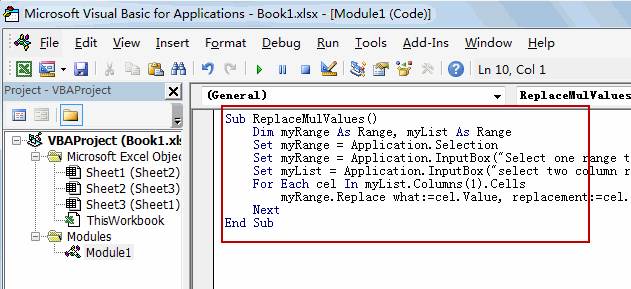```Sub ReplaceMulValues()
Dim myRange As Range, myList As Range
Set myRange = Application.Selection
Set myRange = Application.InputBox("Select one range to be searched", "Find And Replace Multiple Values", myRange.Address, Type:=8)
Set myList = Application.InputBox("select two column range where find/replace pairs are:", "Find And Replace Multiple Values", Type:=8)
For Each cel In myList.Columns(1).Cells
myRange.Replace what:=cel.Value, replacement:=cel.Offset(0, 1).Value
Next
End Sub```

#5 back to the current worksheet, then run the above excel macro. Click Run button.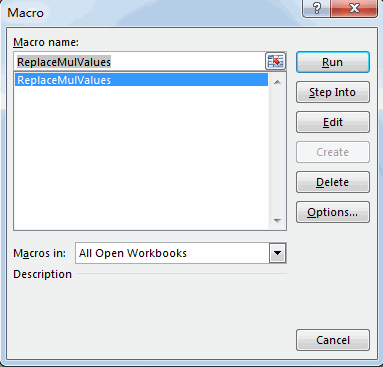#6 Select one range to be searched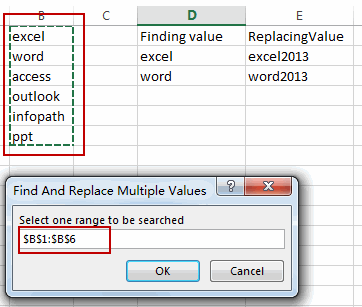#7 select two column range where find/replace pairs are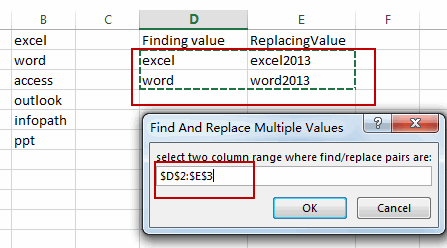#8 let’s see the result.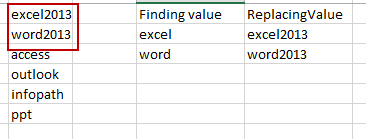### Related Functions

• Excel Substitute function
The Excel SUBSTITUTE function replaces a new text string for an old text string in a text string. The syntax of the SUBSTITUTE function is as below:= SUBSTITUTE  (text, old_text, new_text,[instance_num])….
• Excel IFERROR function
The Excel IFERROR function returns an alternate value you specify if a formula results in an error, or returns the result of the formula.The syntax of the IFERROR function is as below:= IFERROR (value, value_if_error)….
• Excel VLOOKUP function
The Excel VLOOKUP function lookup a value in the first column of the table and return the value in the same row based on index_num position.The syntax of the VLOOKUP function is as below:= VLOOKUP (lookup_value, table_array, column_index_num,[range_lookup])….
• Excel INDEX function
The Excel INDEX function returns a value from a table based on the index (row number and column number)The INDEX function is a build-in function in Microsoft Excel and it is categorized as a Lookup and Reference Function.The syntax of the INDEX function is as below:= INDEX (array, row_num,[column_num])…
• Excel XLOOKUP function
This tutorial will show you how to filter out or extract the top n values from the list having few values using Filter function or XLookup function in Excel The syntax of the xlookup function is as below:=XLOOKUP(lookup value, lookup array, return array, [if not found], [match mode], [search mode])…

## Excel XLOOKUP Function

Excel XLOOKUP Function was added into Excel as a beta feature in August 2019 and is now accessible exclusively in Microsoft 365. (as of July 2021). However, if you fall into this category and often deal with big sets of data in Excel, understanding this method is worthwhile. In this lesson, we will demonstrate how to use the XLOOKUP function to significantly simplify your data search.

## What exactly is Excel XLOOKUP formula?

The Excel XLOOKUP function is a member of the family of lookup and reference functions. It is one of the most helpful features of Microsoft’s famous spreadsheet program. XLOOKUP is the simplest method for finding particular data items inside a cell range. The items included inside a previously set cell range are formatted. Does this sound familiar? The VLOOKUP function likewise operates on this concept. However, the more versatile Excel-XLOOKUP function allows you to look up not just one, but several items. Additionally, you may search for values vertically and horizontally inside your sheet.

In practice, what does this mean? Consider the following scenario: you have a digital client database in the form of an Excel file and are seeking for the address and phone number of a certain individual. With XLOOKUP, you can now search for related items by name and instantly see the needed information. It makes no difference if the sought data are included in a column, row, or table on another page. This indicates that the XLOOKUP function supersedes not only the VLOOKUP but also the HLOOKUP functions.

## XLOOKUP syntax Function

The syntax of the XLOOKUP function is identical to those of VLOOKUP and HLOOKUP. If you’ve ever used them, you’ll appreciate how much more convenient XLOOKUP is. The following is the Excel syntax for the XLOOKUP function:

`=XLOOKUP(lookup value, lookup array, return array, [if not found], [match mode], [search mode])`

The XLOOKUP function accepts up to six parameters, the values of which are shown below.

• `lookup value` (required): the value you’re looking for.
• `lookup array` (required): the array in which the lookup value should be located.
• `return array` (required): the array from which you want to retrieve and return the values when the lookup value is discovered.
• `[if not found]` (optional): If no match is discovered, this value is returned.
• `[match mode]` (optional): This option specifies the sort of match that you are searching for. There are several ways to specify it:
• `0` – It searches for an exact match, and the result must precisely match the lookup array value. When not specified, it is also set as the default.
• `-1` – It searches for an exact match and then returns to the next lower value.
• `1` – It searches for an exact match and then advances to the next bigger number.
• `2` – It performs partial matches using wildcards, where the characters *,?, and have particular significance.

[search mode] (optional): Used to specify the lookup array’s XLOOKUP search mode. There are several ways to specify the same thing:

• 1– Begin the search with the first item. When nothing is supplied, it is defaulted to default.
• -1 – Conducts a reverse search, beginning with the last item.
• 2 – Conducts a binary search in the lookup array in which the data must be sorted ascending. If the data is not properly organized, it may result in mistakes or incorrect outcomes.
• 2 – Conducts a binary search in the lookup array, sorting the data in ascending order. If the data is not properly organized, it may result in mistakes or incorrect outcomes.

XLOOKUP’s Advantages and Disadvantages In Excel, the XLOOKUP function retains some of its benefits over VLOOKUP and INDEX/MATCH. However, it does have certain drawbacks.

1. It operates in both vertical and horizontal directions.
2. Three inputs are required, rather than the four required by the VLOOKUP and INDEX MATCH methods.
3. By default, an exact match is used.
4. Utilize wildcards to conduct partial match lookups.
5. Can execute descending order lookups.

INDEX MATCH now uses a single function rather than two.

## The XLOOKUP Function’s Drawbacks

1. Optional arguments may seem complicated to novices.
2. Can take longer when two ranges are selected and the spreadsheet has an excessive number of cells.
3. When the lookup and array results are not the same length, an error is returned.
4. Both lookup and return ranges must be remembered.

## How to Use Excel’s XLOOKUP Function

The XLOOKUP function is comparable to Excel’s LOOKUP function. XLOOKUP may be used by simply specifying the cell references for the function to act on.

Alternatively, you may utilize the top-level “Formula bar” box to input the XLOOKUP function syntax.

## What makes XLOOKUP superior?

XLOOKUP simplifies and reduces the likelihood of errors in Excel’s most frequently used formulas. Simply type `=XLOOKUP(what you're looking for, the list, the result list)` and you’ll receive the result (or a `#N/A` error if the value is not found).

By default, this function looks for an exact match: One of the drawbacks of VLOOKUP is that you must provide FALSE as the last argument in order to get the right result. XLOOKUP corrects this by defaulting to precise matches. If desired, you may alter the lookup behavior using the match mode option.

The fourth parameter is used to accommodate the value not found situation. To suppress errors in the majority of business cases, we are compelled to encapsulate our lookup formulae with IFERROR or IFNA formulas. XLOOKUP has a fourth argument (described in further detail below) that allows you to choose the default output to use if your value is not found.

XLOOKUP includes extra parameters for searching for unusual circumstances. You may search from the top or bottom of a list, use wildcards, or use quicker alternatives for searching sorted lists.

It outputs a reference, not the value. While this may seem insignificant to casual Excel users, professional Excel users’ eyes light up when they find a formula that returns references. That is, you may mix XLOOKUP results with other formulae in novel ways.

It’s so much cooler to type; all you have to do is type` =XL`. I’m not sure whether this is a lucky coincidence, but saying `=XL` generates this formula.

### Related Functions

• Excel IFERROR function
The Excel IFERROR function returns an alternate value you specify if a formula results in an error, or returns the result of the formula.The syntax of the IFERROR function is as below:= IFERROR (value, value_if_error)….
• Excel VLOOKUP function
The Excel VLOOKUP function lookup a value in the first column of the table and return the value in the same row based on index_num position.The syntax of the VLOOKUP function is as below:= VLOOKUP (lookup_value, table_array, column_index_num,[range_lookup])….
• Excel INDEX function
The Excel INDEX function returns a value from a table based on the index (row number and column number)The INDEX function is a build-in function in Microsoft Excel and it is categorized as a Lookup and Reference Function.The syntax of the INDEX function is as below:= INDEX (array, row_num,[column_num])…
• Excel MATCH  function
The Excel MATCH function search a value in an array and returns the position of that item.The MATCH function is a build-in function in Microsoft Excel and it is categorized as a Lookup and Reference Function.The syntax of the MATCH function is as below:= MATCH  (lookup_value, lookup_array, [match_type])….

## Extract matching values From Two Lists

Suppose that you are working with two lists containing few values, and you want to extract the matching values from those two lists into another separate list. You might prefer to manually extract the matching values from the two lists, which is ok for the few values, but it would be a big deal to extract the matching values from the two lists having multiple cells, and doing it manually would be a foolish attempt because there are 90% chances that you would 100% get tired of it and would never complete your work on time.

But don’t be worry about it because after carefully reading this article, extracting the matching values from the two lists into another separate list would become a piece of cake for you.

So let’s dive into the article to take you out of this fix.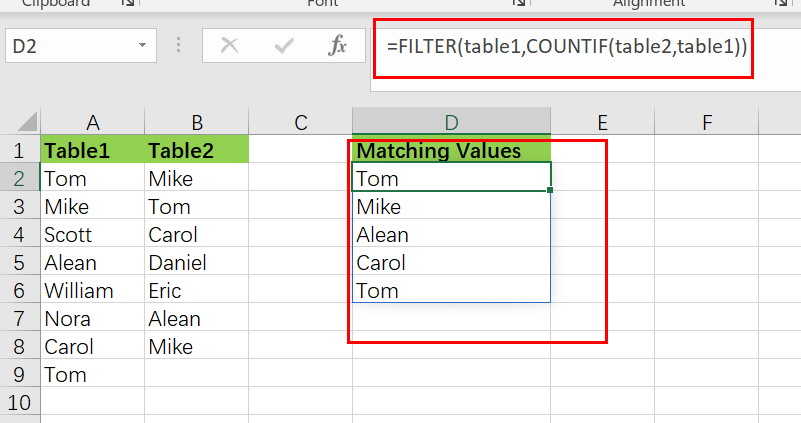## General Formula

The Following Formula would help you compare and extract the matching values from the two lists into another separate list.

`=FILTER(table1,COUNTIF(table2,table1))`

This Formula is based on the FILTER and COUNTIF functions, where the `table1(A2:A9)` and `table2 (B2:B8)` are the named ranges, the list in the `range (D2:D6)` is the list containing the matching values by comparing both table1 and table2.

## Syntax Explanations

Before going into the explanation of the Formula for getting the work done efficiently, we must understand each syntax which would make it easy for you that how each syntax contributes to executing the matching values from the two lists into another separate list:

• `Filter`: This function contributes to narrowing down or filtering out a range of data on the user-defined criteria.
• `COUNTIF`: It is a statistical function that counts the number of cells to meet specific criteria.
• `List`: In this Formula, the list represents the two lists present in the excel worksheet to execute the common values.
• `Comma symbol (,)`: In Excel, this comma symbol acts as a separator that helps to separate a list of values.
• `Parenthesis ()`: The core purpose of this Parenthesis symbol is to group the elements and separate them from the rest of the elements.

## Let’s See How This Formula Works

The FILTER function is used in this Formula to extract data based on a logical test created using the COUNTIF function:

`=FILTER(table1,COUNTIF(table2,table1))`The COUNTIF function is being used to generate the actual filter.

`=COUNTIF(table2,table1))`

It’s worth noticing that we’re using table2 as the range argument and table1 as the criterion argument. In other words, we’re asking COUNTIF to count all values in table1 that occur in table2. We obtain an array with several results since we provide COUNTIF various values for criteria:

`{1;2;0;1;0;0;0;1}`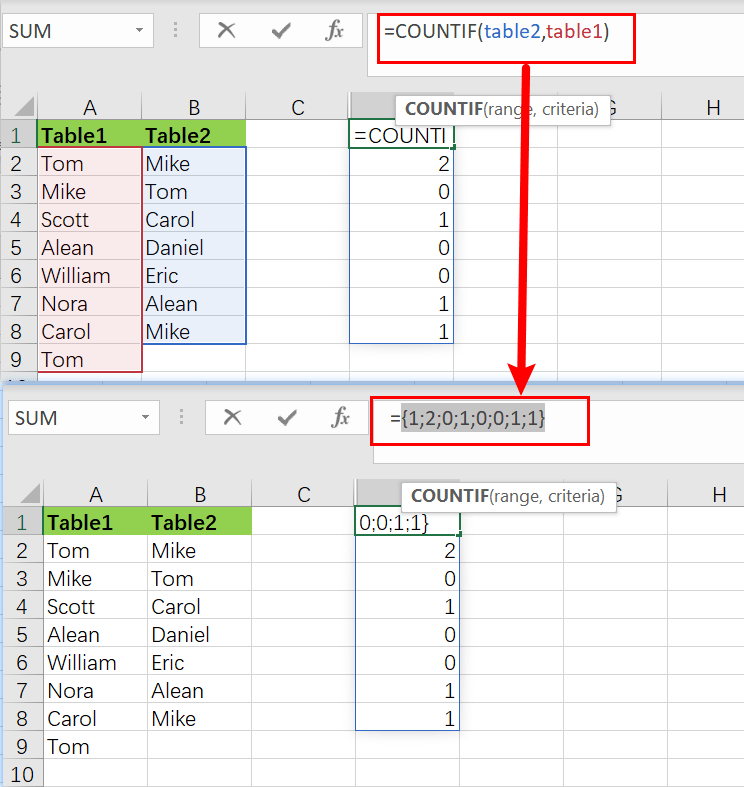Note that the array has 8 counts for each element in the table1. A zero value denotes a value in a table1 that is not present in the table2. Any other positive number denotes a value in table1 that is also present in table2. As the include parameter, this array is passed straight to the FILTER function:

`=FILTER(table1,{1;1;0;1;0;1;0;0;1;0;1;1})`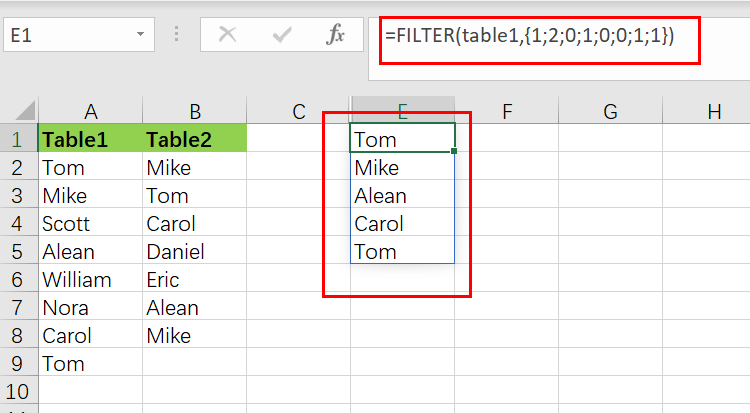The array is used as a filter by the filter function. Any item in the table1 connected with a zero would be eliminated, but any value related to a positive number would retain.

## Extract Non-matching Values

If you want to remove the non-matching values from table1, values in table1 that do not present in table2, we would need to modify the above formula, which is stated as follows:

`=FILTER(table1,NOT(COUNTIF(table2,table1)))`

The NOT function reverses the COUNTIF result, and every non-zero integer returns FALSE, and any zero value returns TRUE. The output is a list of all the values in the table1 that aren’t on the table2.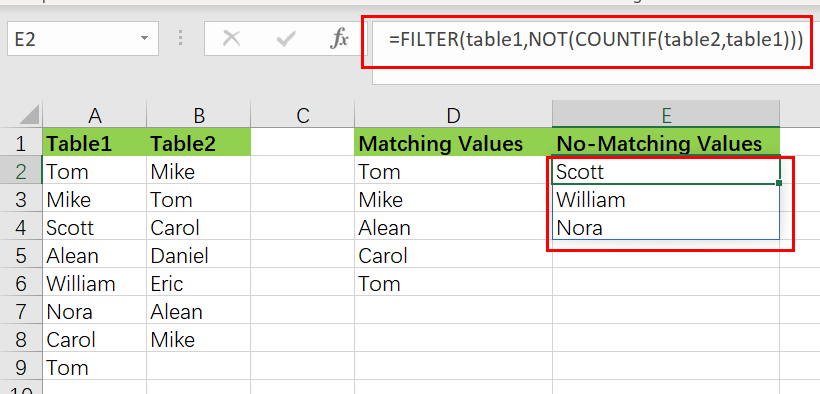## Method two: Extract Matching Values Using INDEX

You can also create a newly formula to extract matching values without the FILTER function, but the Formula would become more complicated. And the formula based on the INDEX function. Like below:

`=IFERROR(INDEX(table1,SMALL(IF(COUNTIF(table2,table1),ROW(table1)-ROW(INDEX(table1,1,1))+1),ROWS(\$D\$2:D2))),"")`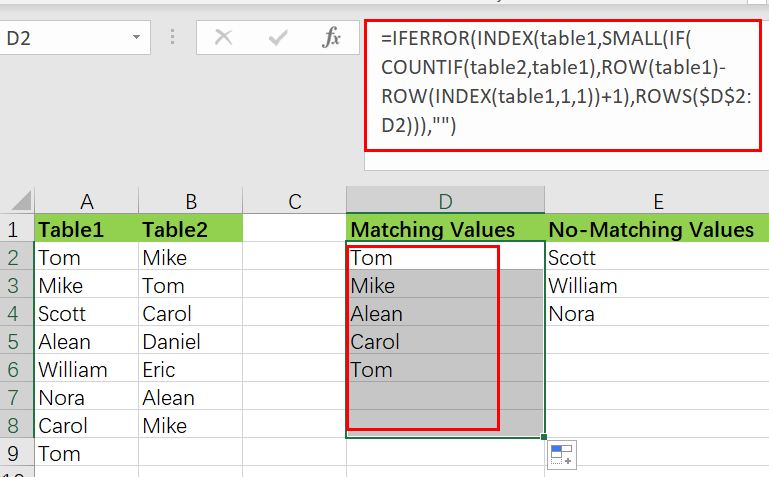Except in Excel 365, this array formula must be typed using `control + shift + enter`.

The INDEX function, which takes table1 as an array parameter, lies at the heart of this Formula. The majority of the remaining Formula determines the row number for matching values. This expression creates a list of relative row numbers as follows:

`=ROW(table1)-ROW(INDEX(table1,1,1)) +1`

which yields a 12-number array reflecting the rows in table1:

`{1;2;3;4;5;6;7;8;}`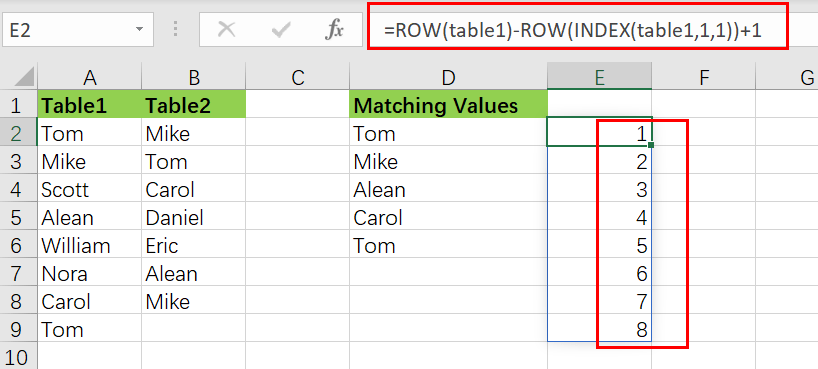These are filtered using the IF function and the same methodology used above in the FILTER Function, but this time using the COUNTIF function:

`=COUNTIF(table2,table1) / identify values that match`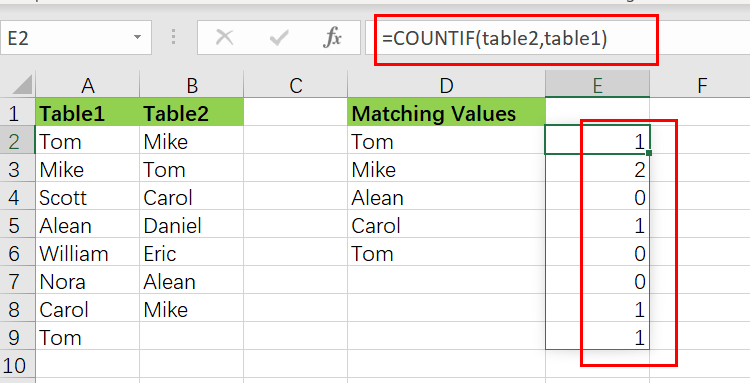`=IF(COUNTIF(table2,table1),ROW(table1)-ROW(INDEX(table1,1,1))+1)`

The resultant array is as follows:

`{1;2;FALSE;4;FALSE; FALSE;7;8; }`

As the Formula is copied along the column, this array is sent immediately to the SMALL function.

The IFERROR function is designed to catch mistakes when a formula is copied down, and the matching values run out.

### Related Functions

• Excel COUNTIF function
The Excel COUNTIF function will count the number of cells in a range that meet a given criteria. This function can be used to count the different kinds of cells with number, date, text values, blank, non-blanks, or containing specific characters.etc.= COUNTIF (range, criteria)…
• Excel Filter function
The FILTER function extracts matched records from a collection of data using one or more logical checks. The include argument specifies logical tests, which might encompass a wide variety of formula conditions.==FILTER(array,include,[if empty])…
• Excel INDEX function
The Excel INDEX function returns a value from a table based on the index (row number and column number)The INDEX function is a build-in function in Microsoft Excel and it is categorized as a Lookup and Reference Function.The syntax of the INDEX function is as below:= INDEX (array, row_num,[column_num])…
• Excel IF function
The Excel IF function perform a logical test to return one value if the condition is TRUE and return another value if the condition is FALSE. The IF function is a build-in function in Microsoft Excel and it is categorized as a Logical Function.The syntax of the IF function is as below:= IF (condition, [true_value], [false_value])….
• Excel ROW function
The Excel ROW function returns the row number of a cell reference.The ROW function is a build-in function in Microsoft Excel and it is categorized as a Lookup and Reference Function.The syntax of the ROW function is as below:= ROW ([reference])….
• Excel SMALL function
The Excel SMALL function returns the smallest numeric value from the numbers that you provided. Or returns the smallest value in the array.The syntax of the SMALL function is as below:=SMALL(array,nth) …
• Excel IFERROR function
The Excel IFERROR function returns an alternate value you specify if a formula results in an error, or returns the result of the formula.The syntax of the IFERROR function is as below:= IFERROR (value, value_if_error)….
• Excel NOT function
The Excel NOT function returns the opposite of a given logical or Boolean value. For example, if you supplied with the value TRUE, the NOT function will return FALSE; If you supplied with the value FALSE, and the NOT function will TRUE. The syntax of the NOT function is as below:=NOT(logical)…

## Excel Filter Function (10 Examples)

This post will guide you how to extracts matched values using FILTER function in Microsoft Excel 365. And also will introduce that how to use FILTER function with same examples in Excel 365.

## Excel Filter Function

The FILTER function “filters” a set of data according to the conditions specified. The outcome is an array of values that match those in the original range. Simply said, the FILTER function extracts matched records from a collection of data using one or more logical checks. The include argument specifies logical tests, which might encompass a wide variety of formula conditions. For instance, FILTER may match data from a given year or month, data containing specific content, or numbers above a specified threshold.

`=FILTER(array,include,[if empty])`

Three parameters are required for the FILTER function: `array`, `include`, and `if empty`.

Where:

• Array – This is required argument. The range or array to filter is specified by array.
• Include – This is required argument. Include one or more logical tests in the include These tests should return TRUE or FALSE depending on the array values evaluated.
• If_empty – This is option argument. The last input, if empty, specifies the value to return if FILTER does not discover any matching values. Typically, this is a message along the lines of “No records found,” although other values may also be returned. To show nothing, provide an empty string (“”).

## Entering FILTER Formula in Excel

FILTER provides dynamic results. When the values in the source data change or the size of the source data array changes, the FILTER results are updated automatically. The results of FILTER will “leak” into numerous cells on the worksheet.

To filter data in Excel using the FILTER function, follow these steps:

Step1: To begin your filter formula, enter `=FILTER(`.

Step2: Enter the address for the range of cells containing the data you want to filter, for example, A2:C10.

Step3: Type a comma, followed by the` filter's condition`, such as `B2:B20>3` (To specify a condition, type the address of the “criteria column,” such as C1:C, followed by an operator symbol such as greater than (>), and finally the criterion, such as the number 3.

Step4: Complete the parenthesis with a closing parenthesis and then hit enter on the keyboard. Your full formula will appear as follows: `=FILTER(A2:C10, B2:B20>3)`

I’ll begin with the fundamentals of utilizing the FILTER function, and then demonstrate some more advanced uses of the FILTER function. This article discusses the FILTER function as a formula entered into spreadsheet cells, not the filter command accessible from the toolbar and pop-up menus.

While using the FILTER function in Excel is almost identical to using it in Google Sheets, there are some subtle variations.

## Excel filtering by a single criteria

To begin, let’s review how to use Excel’s FILTER function in its simplest version, with a single condition/criteria.

I’ll demonstrate how to filter data using a number, a cell value, a text string, or a date… and I’ll also demonstrate how to utilize a variety of “`operators`” in the filter condition (Less than, Equal to, etc…).

### Example 1: How to use a number as a filter

In this first demonstration of how to use the filter tool in Excel, we have a list of students and their grades and wish to create a filtered list of only students with flawless grades.

The assignment: Display a list of students and their grades, but only those who have earned an A.

The reasoning: Filter the range A2:B10 for values larger than 0.7 in the column B2:B10 (70 percent ). Then you can use the following FILTER formula,type:

`=FILTER(A2:B10, B2:B10 >0.7)`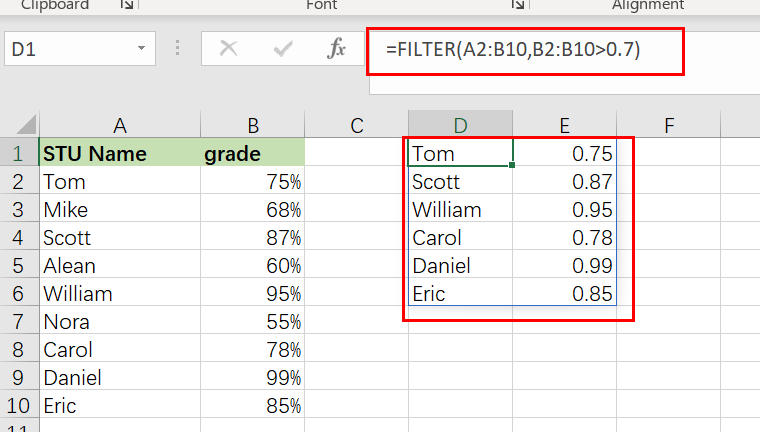### Example 2: How to filter in Excel by a cell value

In this excel filter function example, we want to do the same thing as stated before, but rather than inputting the condition straight into the formula, we’re going to use a cell reference.

When you filter in Excel by a cell value, your sheet is configured in such a way that you may alter the value in the cell at any moment, which updates the value to which the filter criterion is tied.

In this example, rather than explicitly entering the value “0.8” into the formula, the filter criterion is set to cell G1, which contains the “`0.8`” value.

The assignment: Display a list of students and their grades, but only those with a score of less than `80%`.

The reasoning: Filter the range A2:B10 to the extent that B2:B10 is smaller than the value supplied in column G1 (0.8).

You can use the following FILTER formula, type:

`=FILTER(A2:B10, B2:B10 <G1)`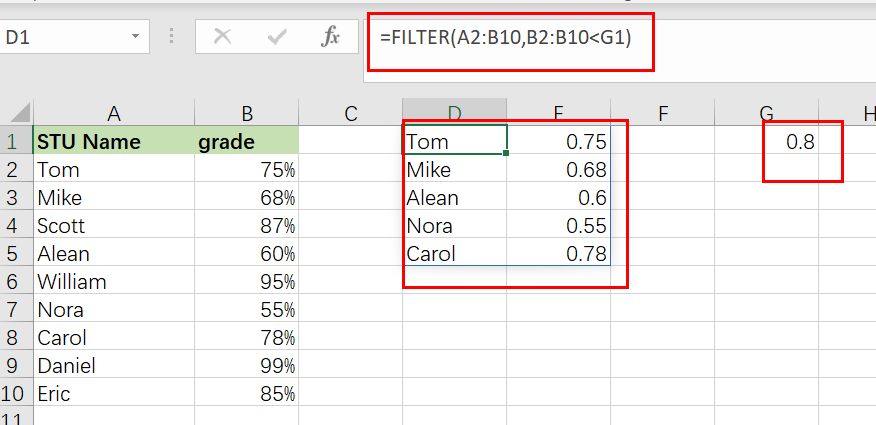### Example 3: Using Excel’s text filter

In this example, we’ll utilize a text string as the filter formula’s criterion. This is fairly similar to using a number, except that the text to filter must be enclosed in quote marks.

We are filtering a list of customers and their payment status in this instance, and we want to present just customers with a payment status of “`Payed`“.

The objective is to provide a list of clients that have paid on their payments.

The reasoning: Filter the range A2:B6 by substituting the string “` Payed` ” for B2:B6.

The following formula: In this example, the formula below is typed in the cell (E1).

`=FILTER(A3:B12, B2:B6=" Payed ")`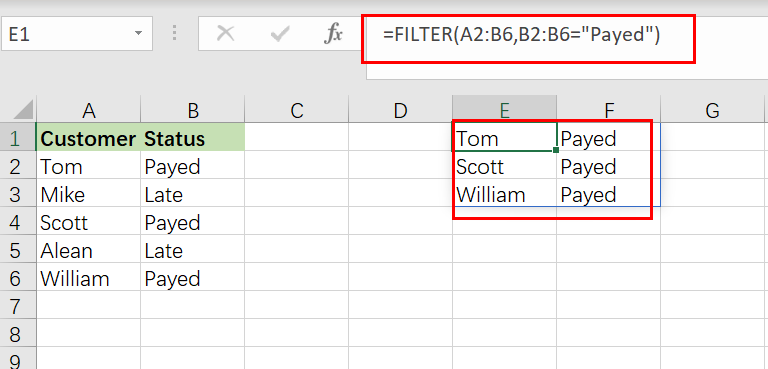### Example 4: Using NOT EQUAL TO as a FILTER condition in Excel

Now that you have a working knowledge of how to use the filter function in Excel, here is another example of filtering by a string of text, but this time we will use the “not equal” operator (<>) to demonstrate how to filter a range and return data that is NOT equal to the criteria you set.

Additionally, we will utilize a bigger data set in this example to show a more comprehensive usage of the FILTER function in the real world.

You may be surprised at how often a circumstance arises in which you need to filter data that is “`not equal to`” a certain number or piece of text.

In this example, we’ll use a report/spreadsheet to display data from sales calls that occur at your organization, and we’ll filter the data to exclude a certain sales person (Scott) from the result.

The assignment: Display sales call statistics for all sales representatives except ” Scott “.

The reasoning: Filter the range `A2:C10` for values `A2:A10` that DO NOT match the string “Scott “.

The following formula: In this example, the formula below is typed in the cell (E1).

`=FILTER(A2:C10, A2:A10 <>"Scott")`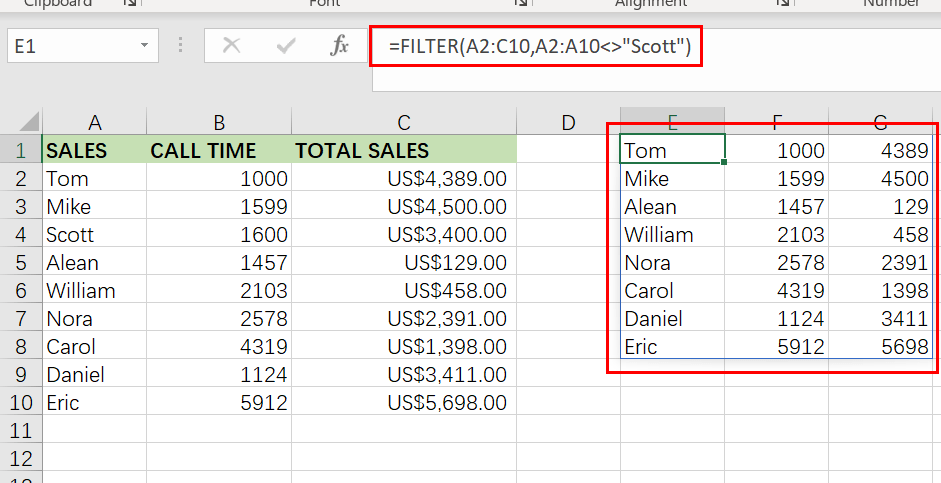Take note that the filtered data on the right side of the figure above does not include any of `Scott `‘s rows/calls.

### Example 5: How to use the date filter in Excel

Filtering in Excel by a date may be accomplished in a few different methods, which I will demonstrate below. If you attempt to put a date into the FILTER function in the same way that you would typically type into a cell, the formula will fail to operate properly.

Therefore, you may either enter the date you want to filter into a cell and then reference that cell in your formula… Alternatively,  you may use the DATE function.

When filtering by date, the same operators (>, =, etc…) are available as they are in other FILTER function applications. Each individual day/date in Excel is merely a number that has been formatted differently. In Excel, for example, the date “`01/30/2022`” is just the serial number “`44591`” formatted as a date. Each time you add a day to the calendar, this number increases by one… For example, “44591” “44592” “44593”

Thus, if one date is farther in the future, it might be regarded “`greater than`” another. In contrast, if one date is farther in the past, it might be considered to be “`less than`” another.

In this example, we’ll use a cell reference to filter on a date. This is identical to the example discussed in Example 2, except that we are dealing with dates instead of percentages.

Consider the following scenario: we want to filter a list of students, their exam results, and the dates on which the tests were administered… and we wish to display only tests conducted before to June (05/01/2022).

`=FILTER(A2:C10, C2:C10 <G1)`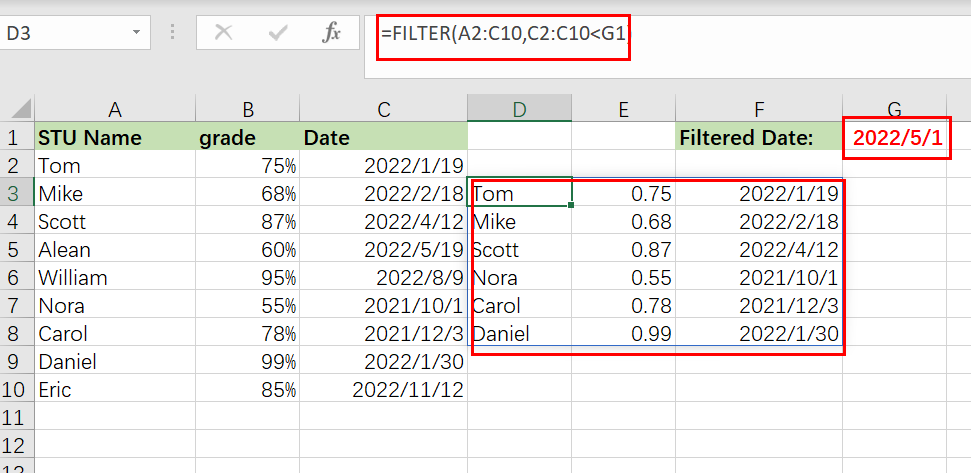### Example 6: Filtering by date in Excel

In this example of date filtering in Excel, we’ll use the same data as in the previous one and attempt to obtain the same results… however, instead of referencing a cell, we’ll utilize the DATE function, which allows you to put the date straight into the FILTER function.

When using the DATE function to provide a date, you must first input the year, followed by the month and finally the day… each denoted with a comma (shown below).

The assignment: Display only exams given before to May

The reasoning: Filter the range `A2:C10 `so that `C2:C10 `is less than or equal to the date (05/01/2022).

The following formula: In this example, the formula below is typed in the cell (D3).

`=FILTER(A2:C10,C2:C10<DATE(2022,5,1))`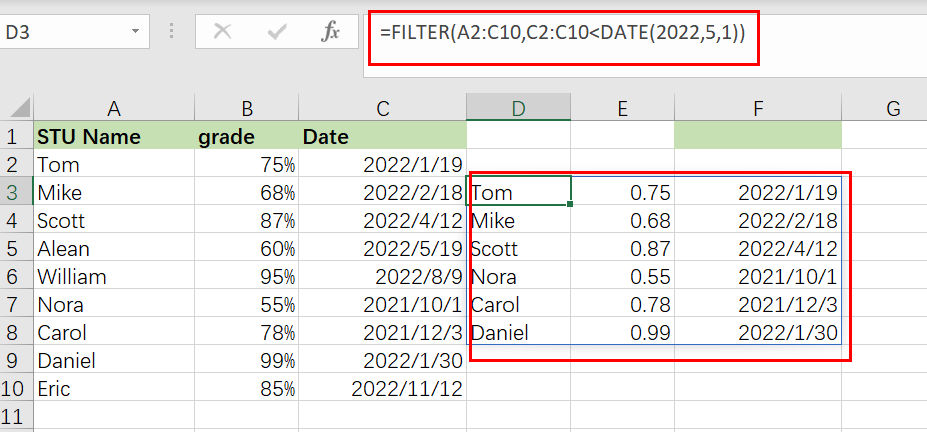### Example 7: Filtering based on two Conditions

When utilizing the Excel FILTER function, you may want to produce data that fits many criteria. I’ll demonstrate two methods for filtering by several criteria in Excel, depending on the scenario and the desired behavior of the calculation.

The conventional method of adding another condition to your filter function (as shown by the Excel formula syntax) allows you to provide a second condition, where both the first AND second conditions must be fulfilled in order for the filter output to be returned.

However, I will demonstrate how to make a little tweak to the function so that you may choose to return/display in the filter function’s output/destination a second condition where EITHER condition might be satisfied. (To utilize AND logic, separate the conditions with an asterisk, or use a plus symbol to separate the criteria.)

In this example, we’re going to filter a collection of data and show those rows that satisfy BOTH the first and second conditions.

To utilize a second condition in this manner (using AND logic), just insert it after the first condition in the formula, separated by an `asterisk (*)`. Each condition must be included in a separate pair of parentheses.

When a filter formula is used with several conditions, the columns referenced in each condition must be distinct.

In this case, we’d want to filter a list of clients based on their payment status and region… and to display those customers who are both current members AND paid on their payment status.

The objective is to provide a list of customers who are paid on payments, but only those who are in East region.

The reasoning: Filter the range A2:C6 such that B2:B6 equals the text “`Paid`,” AND C2:C6 equals the text “`East`“.

The following formula: In this example, the formula below is typed in the blue cell (E1).

`=FILTER(A2:C6,( B2:B6="Paid")*( C2:C6 ="East"))`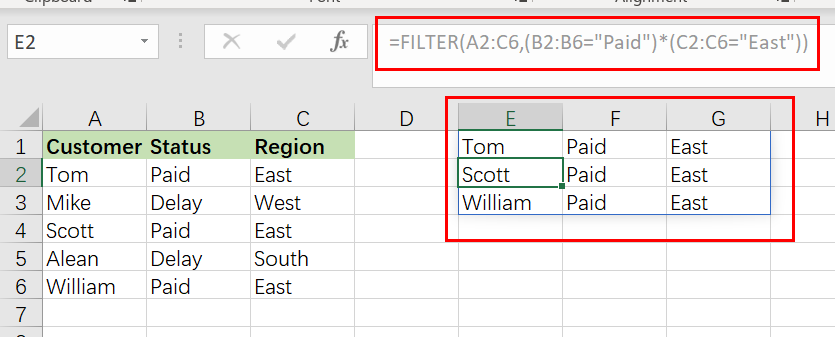### Example 8: Filtering Based on Two Conditions using OR Logic

In this example, we’re going to filter a collection of data and show those rows that satisfy either the first OR the second criterion.

To utilize a second condition in this manner (using OR logic), just insert it after the first condition in the formula, separated by a `plus sign`. Each condition must be included in a separate pair of parentheses (shown below).

When used in this manner, the FILTER formula allows you to choose criteria from the same or separate columns.

In this case, we’re filtering the same customer data as in the previous example, but this time we’re displaying a list of customers who either are in `East` region OR have paid on a payment. This will generate a list of clients to whom a payment notification have paid… whether they are current members or east region who have paid on their last payment.

The objective is to provide a list of customers who are in `East` region, as well as customers who have paid on payments regardless of whether they are in East region.

The following formula: In this example, the formula below is typed in the cell (E1).

`=FILTER(A2:C6, (B2:B6="Paid ")+(C2:C6=" East ")`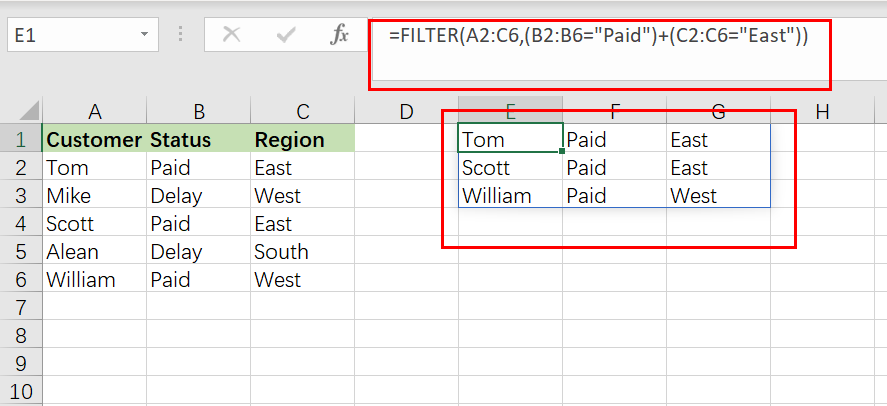### Example 9: Filter Data in Excel from Another Sheet

You may often encounter instances in Excel when you need to filter data from another sheet, where your raw unfiltered data is on one tab and your filter formula is on another sheet.

This may be accomplished by simply referring to a certain sheet’s name when providing the filter’s ranges. Thus, while you would typically give a range such as “A1:B4,” when referring another sheet when filtering, you indicate the sheet name by preceding the range with the sheet name and an exclamation mark, as in “`SheetName!A1:B4`“.

However, if the sheet name contains a space, an apostrophe must be used before and after the sheet name, as in `"Sheet Name!" A1:B4`.

The following is an example of how to filter data in Excel from a separate sheet, where the filter formula is located on a different sheet than the source range.

Consider the following scenario: On one sheet, you have a list of customers and their payment status, and you wish to present a filtered list of  paid customer on another sheet.

The job is to filter the list of customers on the Sheet3 and to display a separate list of customer names with a pay status on another worksheet.

The reasoning: Filter the range using the `Sheet3` command! A2:C6, where ‘ Sheet3′ is the range! B2:B6 corresponds to the phrase “Paid“.

The following formula: In this example, the formula below is typed in the cell (A3).

`=FILTER(Sheet3! A2:C6, Sheet3!B2:B6="Paid")`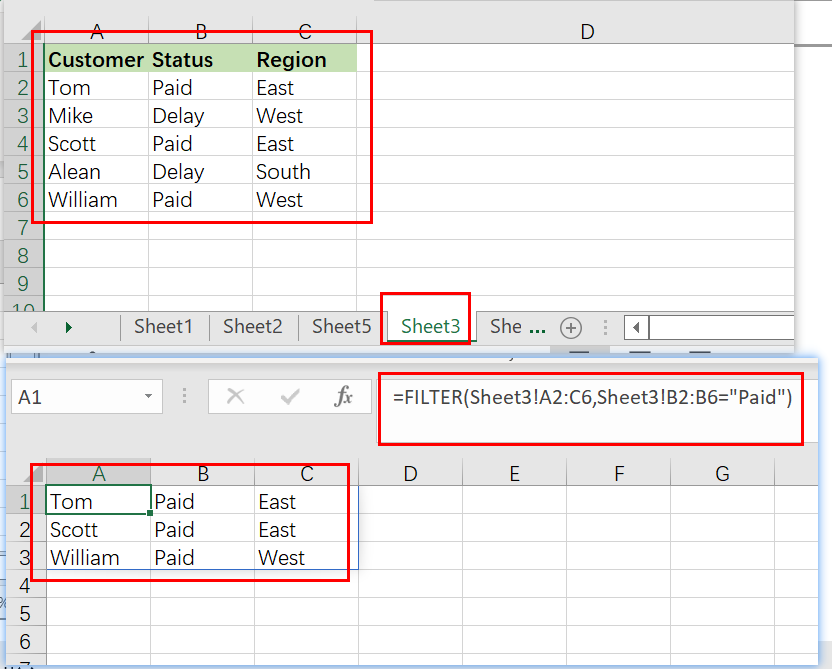### Example 10: Providing Maximum Number of Rows of Filtered Data

If your FILTER formula provides a large number of rows but your worksheet is restricted in space and you are unable to erase the data below, you may limit the amount of rows returned by the FILTER function.

Let us demonstrate how it works using a simple formula that filter data that grade is less than 0.7 from filter value in Cell F1:

`=FILTER(A2:C10, B2:B10<F1)`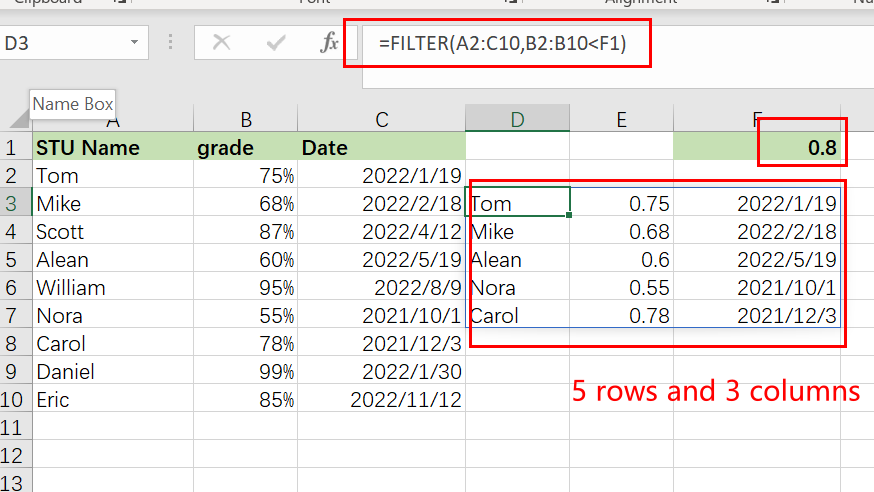The preceding formula produces all records that it discovers, in this instance five rows. However, imagine you only have room for two. To output just the first two rows discovered, follow these steps:

Step1: Incorporate the FILTER formula into the INDEX function’s array parameter

Step2: Use a vertical array constant such as 1;2 as the row num input to INDEX. It specifies the number of rows to return (2 in our case).

Step3: Use a horizontal array constant such as 1,2 for the column num parameter. It defines the columns that should be returned (the first 2 columns in this example).

Step4: To account for any mistakes caused by the absence of data meeting your criteria, you may wrap your calculation in the IFERROR function.

The entire excel filter formula is as follows:

`=IFERROR(INDEX(FILTER(A2:C10, B2:B10<F1), {1;2},{1,2}), "No Found")`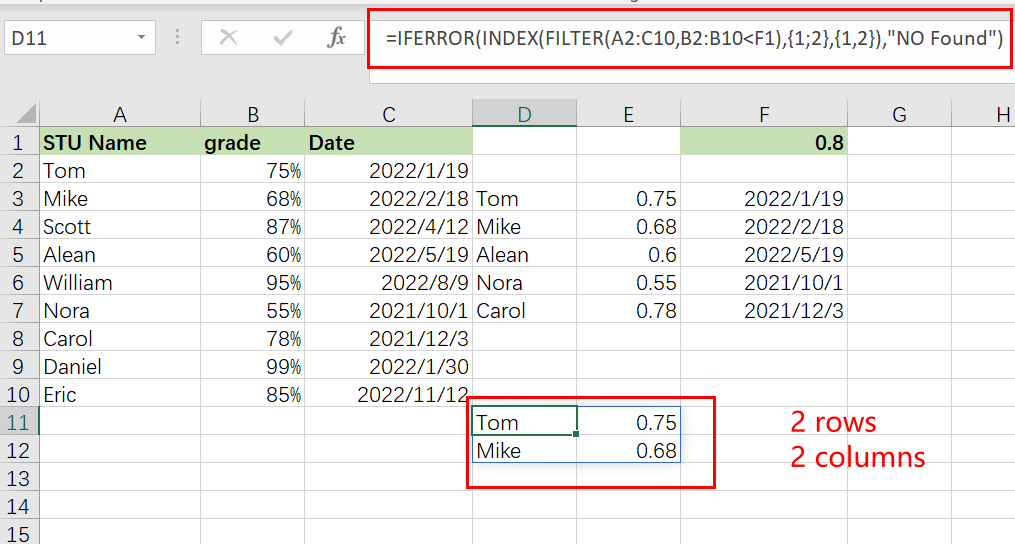## Conclusion

This section discusses the FILTER function and its many uses. In general, when it comes to time management, we need this feature for a variety of reasons. I demonstrated various techniques with accompanying examples, however there might be countless further iterations based on a variety of circumstances. If you know of another way to use this function, please share it with us.

### Related Functions

• Excel IFERROR function
The Excel IFERROR function returns an alternate value you specify if a formula results in an error, or returns the result of the formula.The syntax of the IFERROR function is as below:= IFERROR (value, value_if_error)….
• Excel INDEX function
The Excel INDEX function returns a value from a table based on the index (row number and column number)The INDEX function is a build-in function in Microsoft Excel and it is categorized as a Lookup and Reference Function.The syntax of the INDEX function is as below:= INDEX (array, row_num,[column_num])…

## Extract Multiple Match Values into Separate Columns

If you have a few values/items in the excel sheet and you are thinking that with the aid of the “VlOOKUP” function you can look for a specific value, extract it and then put the matching item into the separate column in Ms Excel easily, then congratulations, you are thinking right, but here a problem arises that there isn’t any doubt that by this way you can extract one or two matches into the separate column easily but with the aid of this way you cannot extract multiple matches into separate columns and if you would do that by this way then there are 90% chances that you would 100% get tired of it and can’t complete your task at the right time.

But don’t be worry about it because after carefully reading this article extracting multiple matches into the separate columns would become a piece of cake for you.

So let’s dive into the article to take you out of this fix.

## General Formula:

For extracting multiple matches(items) into seprate columns you can use the  Array Formula which is based upon INDEX and SMALL, which is stated as follows:

`=IFERROR(INDEX(STU_Range,SMALL(IF(CLASS_Range=\$E2, ROW(STU_Range) -MIN (ROW(STU_Range))+1),COLUMNS(\$E\$2:E2))),"")`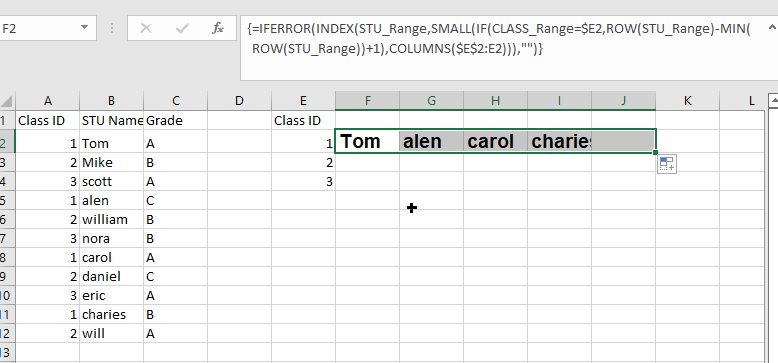## Syntax Explanations:

Before knowing about how to use this formula for getting the work done efficiently, we must understand each syntax which would make it easy for you that how each syntax contributes to extracting multiple matches into the separate columns:

• `IFERROR`: This Function returns a custom result whenever a formula generates an error and returns the expected result when no error is detected.
• `INDEX`: In a range or array, this index function contributes to returning the value at a given position.
• `SMALL`: From the given range of data, this small Function returns the Nth
• `IF`: In Excel, this IF Function contributes to returning two different values, one value for the TRUE result and another for the FALSE result.
• `ROW`: In Excel, this Row function contributes toreturning the row number as a reference.
• `MIN`: From the range of input values, this MIN function contributes to returning the smallest numeric value.
• `Absolute Reference`: The Absolute referenceis nothing but an actual fixed location in a worksheet.
• `COLUMNS`: From a given reference, this Column function contributes to counting the columns.
• `Comma symbol (,)`: This symbol acts as a separator that contributes to separating a list of values.
• `Minus Operator (-)`: This minus symbol contributes to subtracting any two values.
• `Parenthesis ()`: The primary purpose of this parenthesis symbol is to group the various elements.
• `Name`– It represents the input ranges in your worksheet.
• `Plus operator (+)`: This plus symbol adds the values.

## Let’s See How This Formula Works:

To use this array formula for getting the work done, you must enter this formula with Control + Shift + Enter. As soon as you would enter this formula into the first cell, you need to drag it down and across to fill in the other cells.

As you can see in the above screenshot, this formula uses two names ranging: “ CLASS_Range ” and “ STU_Range,” where “ STU_Range ” refers to B2:B12 and on the other hand “ CLASS_Range ” refers to A2:A12.

You would definitely wonder that how this formula works to extract multiple matches into columns? So here is the answer. In this formula, we use the Small Function and INDEX function, which work together.

As the SMALL Function (dynamically constructed by IF) is used to obtain row number corresponding to an “nth match,” so after getting the row number from SMALL Function, this would then pass it into the INDEX function, which returns the value at that row, this is also the main motive of this formula.

The snippet “IF(CLASS_Range=\$E2, ROW(STU_Range) -MIN (ROW(STU_Range))+1” tests the named range “ STU_Range ” for the value in E2. If the value is found, then from an array of relative row numbers, it would return a row number, which is created with:

`=ROW(STU_Range) -MIN (ROW(STU_Range))+1`

The output of this formula is :

`{1;2;3;4;5;6;7;8;9;10;11}`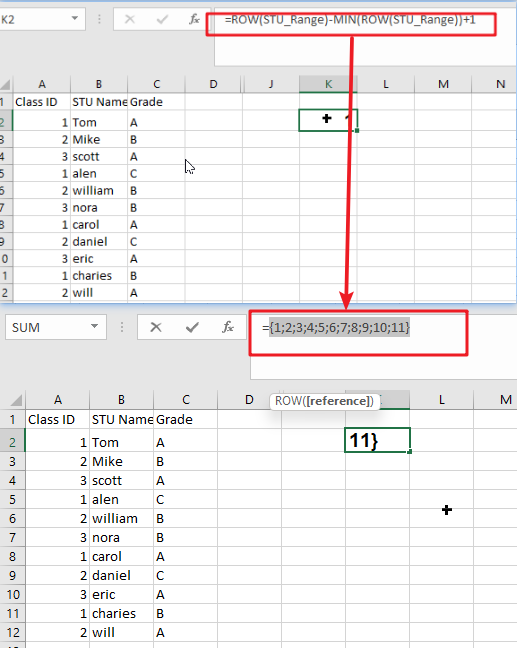Now the final result is an array that would contain the numbers where there is a match, and FALSE where there is not any match found:

`{1;FALSE;FALSE;4;FALSE;FALSE;7;FALSE;FALSE;10;FALSE}`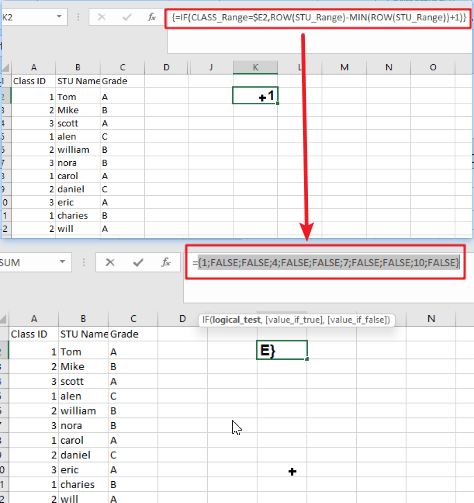Then this array goes into the SMALL Function. By expanding range(Given Below), The k value for SMALL (nth) returns:

`COLUMNS(\$E\$2:E2)`

The SMALL function returns each matching row number, which is then supplied as the row_num to the INDEX function as the array with the range named “ STU_Range.”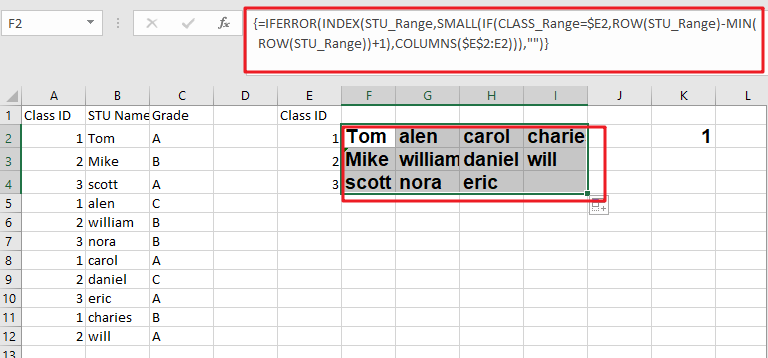Notes:

Now, this question would pop up in your mind that how would it handle the errors? Then whenever the COLUMN would return a value for k that does not exist, the #NUM error would be thrown by the SMALL Function at the next moment. This usually occurs when all the matches have occurred. To tackle the errors, the formula is wrapped up in the Function named “IFERROR,” which would receive the errors and then return an empty string (” “).

### Related Functions

• Excel INDEX function
The Excel INDEX function returns a value from a table based on the index (row number and column number)The INDEX function is a build-in function in Microsoft Excel and it is categorized as a Lookup and Reference Function.The syntax of the INDEX function is as below:= INDEX (array, row_num,[column_num])…
• Excel MATCH  function
The Excel MATCH function search a value in an array and returns the position of that item.The MATCH function is a build-in function in Microsoft Excel and it is categorized as a Lookup and Reference Function.The syntax of the MATCH function is as below:= MATCH  (lookup_value, lookup_array, [match_type])….
• Excel IF function
The Excel IF function perform a logical test to return one value if the condition is TRUE and return another value if the condition is FALSE. The IF function is a build-in function in Microsoft Excel and it is categorized as a Logical Function.The syntax of the IF function is as below:= IF (condition, [true_value], [false_value])….
• Excel ROW function
The Excel ROW function returns the row number of a cell reference.The ROW function is a build-in function in Microsoft Excel and it is categorized as a Lookup and Reference Function.The syntax of the ROW function is as below:= ROW ([reference])….
• Excel SMALL function
The Excel SMALL function returns the smallest numeric value from the numbers that you provided. Or returns the smallest value in the array.The syntax of the SMALL function is as below:=SMALL(array,nth) …
• Excel MIN function
The Excel MIN function returns the smallest numeric value from the numbers that you provided. Or returns the smallest value in the array.The MIN function is a build-in function in Microsoft Excel and it is categorized as a Statistical Function.The syntax of the MIN function is as below:= MIN(num1,[num2,…numn])….
• Excel IFERROR function
The Excel IFERROR function returns an alternate value you specify if a formula results in an error, or returns the result of the formula.The syntax of the IFERROR function is as below:= IFERROR (value, value_if_error)….
• Excel COLUMN function
The Excel COLUMN function returns the first column number of the given cell reference.The syntax of the COLUMN function is as below:=COLUMN ([reference])….

## VLOOKUP From Another Sheet Not Working

In the previous post, you should know that how to fix or remove the #N/A error when using VLOOKUP formula to lookup value from another sheet. And this post will show you reasons why your VLOOKUP formula is not working in Excel 2003/2010/2013/2016 or Excel 365. You should be want to know some of the common reasons why you are getting those VLOOKUP errors, such as:  #N/A, #VALUE, #REF, and returning incorrect results.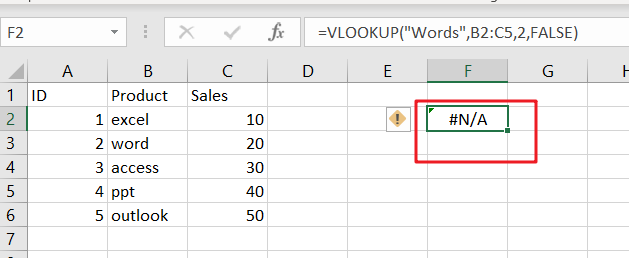## Most of Common VLOOKUP Formula Errors

You should know that VLOOKUP function is a very popular and powerful used function in Microsoft Excel or Google Sheets. And most of users are also complain that the VLOOKUP function fails to work sometimes, and want to know how to fix any VLOOKUP not working problem while using VLOOKUP function to lookup value from another sheet in Excel. Let’s see some common VLOOKUP Formula errors below:

### 1. VLOOKUP #N/A Error

When using VLOOKUP formula to lookup one value in your table array, and if it cannot find an exact match or approximate match, then the #N/A error message would be displayed.  #N/A error means “The Value is not available” in Excel. this error is the most common VLOOKUP error message while looking up values from another sheet or different sheet and returns an incorrect value.

It is not only one reason that the lookup value is not available, and there should be some reasons whey VLOOKUP formula is not working and returns errors in Excel.

#1 Lookup Value Not in the First Column

For VLOOKUP function, the Lookup value must be in the first column or the leftmost column of the table_array argument. If lookup value is not available in the first column of a table array, and the VLOOKUP formula will generate an #N/A error.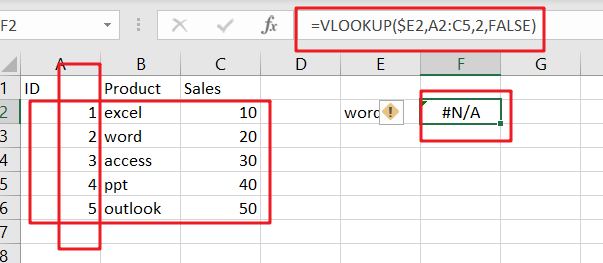If you want to fix this VLOOKUP error in your worksheet, and you need to re-arrange your column range correctly in your table array parameter in VLOOKUP formula. for the above VLOOKUP formula example, you need to change your table_array range from A2:C5 as B2:C5 in VLOOKUP function.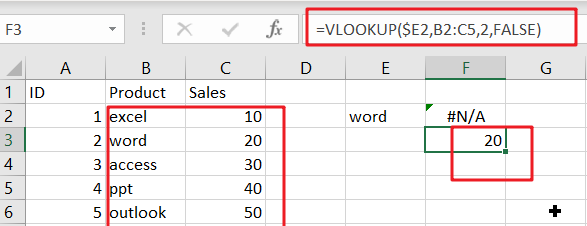#2 Using an Approximate Match

The range_lookup is an optional argument in the VLOOKUP function. And the value can be set as True or False. The default value is True. If you don’t specify this argument and Excel will assume that you want to use an approximate match. And if you want to use an Exact Match in VLOOKUP function, and you should set the fourth argument as FALSE.

Assume that the range_lookup argument is set to “FALSE” or using an approximate match in your VLOOKUP formula, if you want to lookup value from another sheet or same sheet using VLOOKUP function, and lookup value is smaller than the smallest value available in the first column of table array, and your VLOOKUP function should be generated an #N/A error.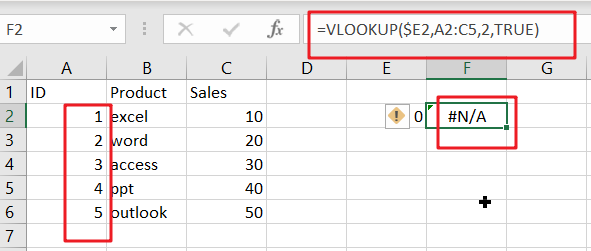#3 Numbers Stored or Formatted as Text

When your data is imported from an external data table or your numeric values were stored as text format, and you try to lookup a numeric value using VLOOKUP function, Excel will generate an error message #N/A.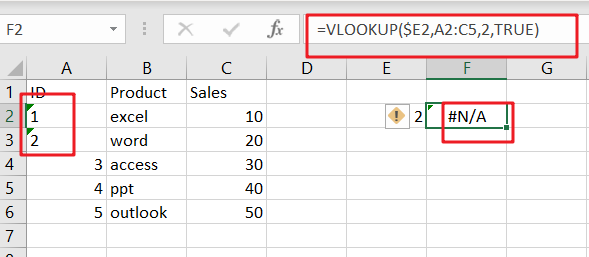If you want to fix this error message for VLOOKUP formula, and you have to check and format all numeric values as Number format. you can select those values, and go to “HOME” tab, and select “Number” format from the drop-down list in the Number category.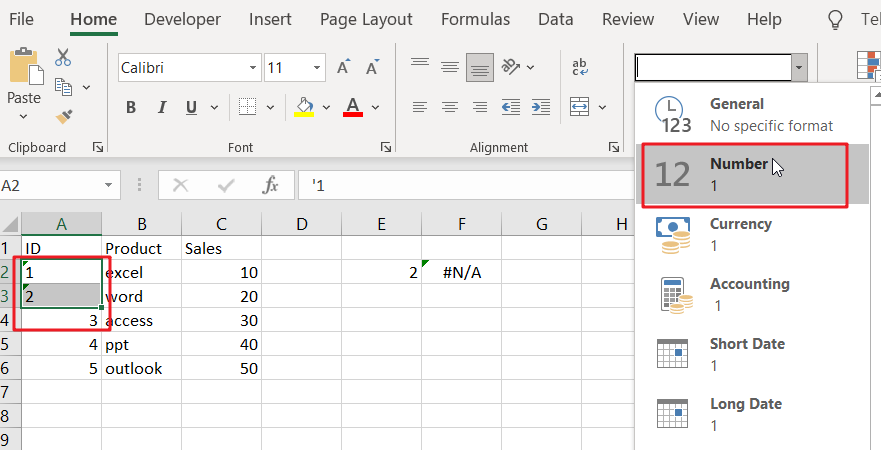#4 Type Mistake for Lookup Value

The VLOOKUP function will use the lookup value as keyword to search for in the lookup table in your current worksheet or in another worksheet, if you mistyped the lookup value and the VLOOKUP function will not find what you typed and Excel will return you an error message #N/A.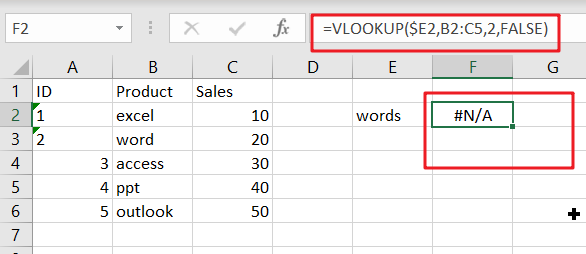For example, if you want to type the lookup value “word”, but mistyped as “words”, and the VLOOKUP function would display the #N/A error message. When the keyword was typed correctly, and the formula should be able to find the expected value.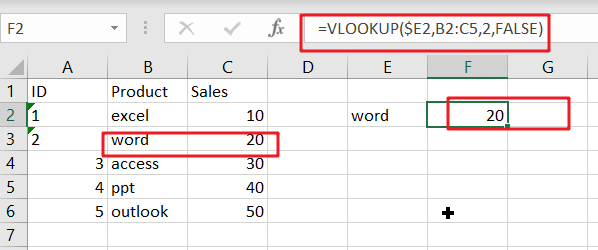#5 Extra Space or Characters (Leading and trailing spaces for Lookup value)

If the lookup value contains extra space or characters in the formula, and the VLOOKUP function will also generate an error message #N/A. the #N/A error is caused by extra spaces which your eyes can hardly see, and everything looks good.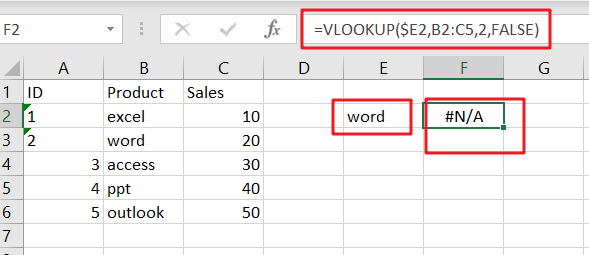To fix or remove this error, and you should check for extra spaces in the formula or remove leading and trailing spaces in the lookup value using TRIM function.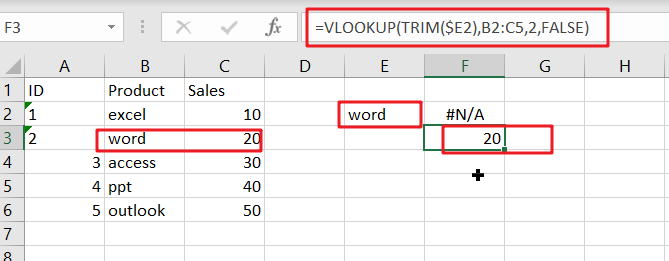### 2. VLOOKUP #REF error

#1 Count the Wrong column number for Column_index_num parameter

If you count the wrong column number for Column index number parameter in the third argument of the VLOOKUP function, and it will also generate an error message #N/A. To fix this error, you need to recount the COLUMNS count from the table array that you are getting your data in your VLOOKUP formula.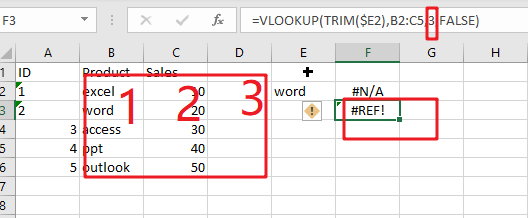### 3. VLOOKUP #VALUE Error

If you enter wrong data type in the VLOOKUP formula in Excel, and the #VALUE error will generate. And the below reasons will also cause this error.

• Index Number is less than 1

If your Index_number argument is less than 1 in VLOOKUP function, and it will return a #VALUE error.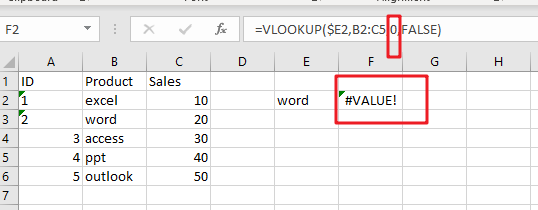• Lookup Value Length

You need to know that lookup value length should not be exceeded 256 characters, if lookup value character length exceeds this limit in VLOOKUP formula, and it returns a #VALUE error.

To fix this error, you can use INDEX function in combination with MATCH function to build another newly formula to achieve the same request.

### 4. Removing VLOOKUP Error Messages

If you want to make VLOOKUP errors clearer and easier to understand, and you can use the IFERROR function to display a meaningful message if VLOOKUP is not working and returns error.

`=IFERROR(VLOOKUP(\$E2,Sheet8!B2:C5,2,FALSE),"lookup value not found!!!")`

The IFERROR function can be used to check for any errors in the VLOOKUP formula, and if one VLOOKUP error message is returned by the VLOOKUP formula, excel will display “lookup value not found!!!” instead of any of the error messages, such as: #N/A,#REF,and #VALUE.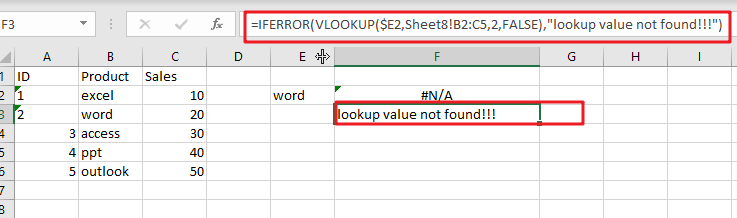Enjoy!

### Related Functions

• Excel IFERROR function
The Excel IFERROR function returns an alternate value you specify if a formula results in an error, or returns the result of the formula.The syntax of the IFERROR function is as below:= IFERROR (value, value_if_error)….
• Excel VLOOKUP function
The Excel VLOOKUP function lookup a value in the first column of the table and return the value in the same row based on index_num position.The syntax of the VLOOKUP function is as below:= VLOOKUP (lookup_value, table_array, column_index_num,[range_lookup])….
• Excel INDEX function
The Excel INDEX function returns a value from a table based on the index (row number and column number)The INDEX function is a build-in function in Microsoft Excel and it is categorized as a Lookup and Reference Function.The syntax of the INDEX function is as below:= INDEX (array, row_num,[column_num])…
• Excel MATCH  function
The Excel MATCH function search a value in an array and returns the position of that item.The MATCH function is a build-in function in Microsoft Excel and it is categorized as a Lookup and Reference Function.The syntax of the MATCH function is as below:= MATCH  (lookup_value, lookup_array, [match_type])….

## Fix #N/A Error For VLOOKUP From Another Sheet

This post will show you how to fix the #N/A error why it occurs when you extract values from another sheet using VLOOKUP function in Excel 2016,2013,2010 or other Excel versions. How can you correct a #N/A error in VLOOKUP function in Excel. This post will also show you several reasons why VLOOKUP formula is not working and displaying the #N/A, #NAME and #VALUE error messages.

## The Reasons Why VLOOKUP Formula is Not Working

#1 Lookup value is not in the first column of the table array

The VLOOKUP function is that it can only look for values on the left-most column in the table array and if lookup value is not in the first column of the array, and you will see the #N/A error.

Assume that you want to retrieve the number of sales for product “word”, and you can use the following VLOOKUP function:

`=VLOOKUP(E2,A2:C6,2,FALSE)`

You should see that the #N/A error message will be shown, because the lookup value “word” in Cell E2 appears in the second column of the table array argument A2:C6.

To correct the #N/A error, and you need to adjust your VLOOKUP to reference the correct column.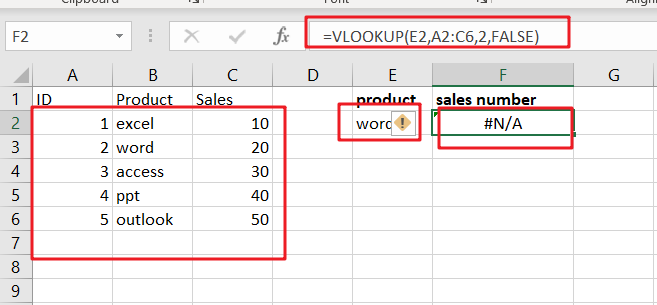#2 #N/A Error in Exact Match VLOOKUP

If you are starting with an exact match and the exact value “Words” is not found, and the #N/A error is returned. Type the following VLOOKUP formula in Cell F2:

`=VLOOKUP("Words",B2:C5,2,FALSE)`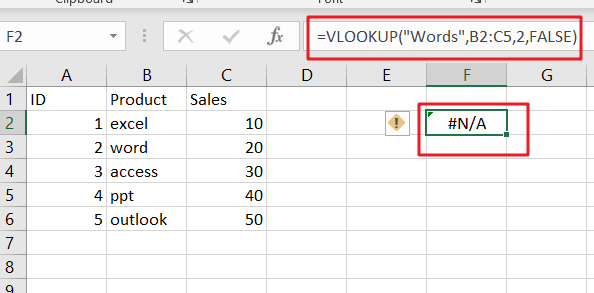#3 #N/A Error in Approximate Match VLOOKUP

If you are starting with an approximate match using VLOOKUP function, and your formula may be return the #N/A error message caused by the following two cases:

If the lookup column is not sorted in ascending order.

If the lookup value is smaller than the smallest value in the lookup array.

## Remove VLOOKUP #N/A Error

When using VLOOKUP function and it can not find any data or when you haven’t entered a lookup value in VLOOKUP formula, and you should get an #N/A error message. And this error message is not helpful at all. You may be want to remove it in your worksheet. And you can use the IFERROR function to remove #N/A error message in VLOOKUP function.

You can put the above VLOOKUP function into the IFERROR function as a new formula, refer to the below formula based on the IFERROR function and VLOOKUP function, it returns a blank string instead of #N/A error.

`=IFERROR(VLOOKUP("Words",B2:C5,2,FALSE),"")`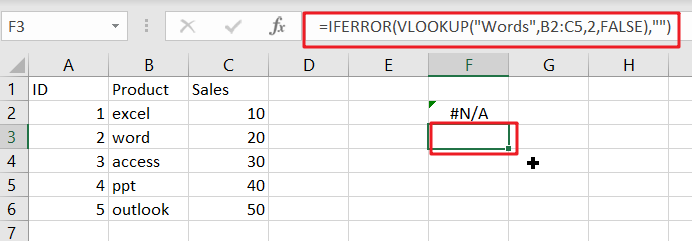If you are using Excel 2003, and you can use the IF function and the ISERROR function, as the IFERROR function is not available in Excel 2003, type:

`=IF(ISERROR(VLOOKUP("Words",B2:C5,2,FALSE)),"",VLOOKUP("Words",B2:C5,2,FALSE))`

You need to put the VLOOKUP function in there twice to remove #N/A error message in Excel 2003.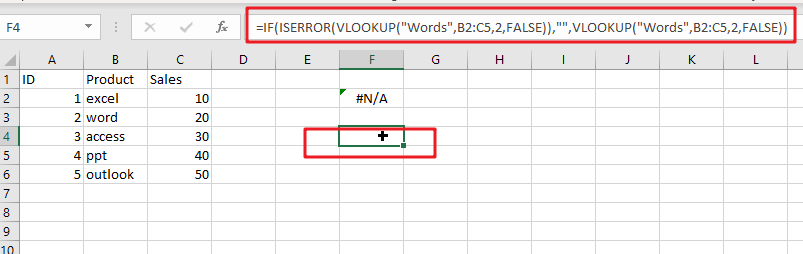### Related Functions

• Excel IF function
The Excel IF function perform a logical test to return one value if the condition is TRUE and return another value if the condition is FALSE. The IF function is a build-in function in Microsoft Excel and it is categorized as a Logical Function.The syntax of the IF function is as below:= IF (condition, [true_value], [false_value])….
• Excel ISERROR function
The Excel ISERROR function used to check for any error type that excel generates and it returns TRUE for any error type, and the ISERR function also can be checked for error values except #N/A error, it returns TRUE while the error is #N/A. The syntax of the ISERROR function is as below:= ISERROR (value)….
• Excel IFERROR function
The Excel IFERROR function returns an alternate value you specify if a formula results in an error, or returns the result of the formula.The syntax of the IFERROR function is as below:= IFERROR (value, value_if_error)….
• Excel VLOOKUP function
The Excel VLOOKUP function lookup a value in the first column of the table and return the value in the same row based on index_num position.The syntax of the VLOOKUP function is as below:= VLOOKUP (lookup_value, table_array, column_index_num,[range_lookup])….

## How to Count Duplicate Values Only Once in A Range in Excel?

When counting the number of times for objects appear in a list or a range, we usually record the duplicate value only once and ignore the redundant ones. We cannot apply formula with only one function to implement this in excel, so we need to update formula and use a formula combine more than one functions. This free tutorial will introduce you to count objects only once by the combination of SUMPRODUCT and COUNTIF functions.

Precondition:

Insert a list with some products. You can see there are some duplicate values. We will introduce you the method to count numbers ignore the redundant duplicate values. Actually, for case-sensitive and case-insensitive two different situations, we provide two ways with two different formulas to count numbers.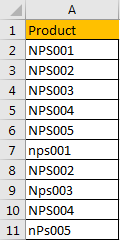## Part 1: Count Duplicate Values Once with Case Sensitive

Step 1: Select a blank cell just next to the list, for example B2, enter the formula =SUM(IFERROR(1/IF(A2:A11<>””, FREQUENCY(IF(EXACT(A2:A11, TRANSPOSE(A2:A11)), MATCH(ROW(A2:A11), ROW(A2:A11)), “”), MATCH(ROW(A2:A11), ROW(A2:A11))), 0), 0)).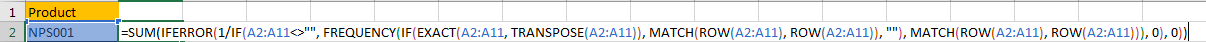Step 2: Press Shift+Ctrl+Enter on keyboard simultaneously to get result.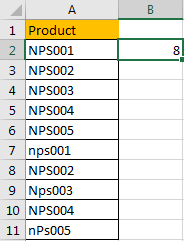As we count number with case sensitive in this sample, so product ‘NPS001’ and ‘nps001’ are processed as two different products.

## Part 2: Count Duplicate Values Once with Case Insensitive

This time we count number with case insensitive.

Step 1: In B2 enter the formula =SUMPRODUCT((A2:A11<>””)/COUNTIF(A2:A11,A2:A11&””)).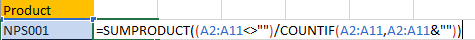Step 2: Press Enter directly to get result. Verify that this time the count number is 5, so this time products ‘NPS001’ and ‘nps001’ are considered as duplicate values.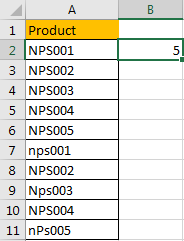### Related Functions

• Excel SUMPRODUCT function
The Excel SUMPRODUCT function multiplies corresponding components in the given one or more arrays or ranges, and returns the sum of those products.The syntax of the SUMPRODUCT function is as below:= SUMPRODUCT (array1,[array2],…)…
• Excel COUNTIF function
The Excel COUNTIF function will count the number of cells in a range that meet a given criteria. This function can be used to count the different kinds of cells with number, date, text values, blank, non-blanks, or containing specific characters.etc.= COUNTIF (range, criteria)…
• Excel SUM function
The Excel SUM function will adds all numbers in a range of cells and returns the sum of these values. You can add individual values, cell references or ranges in excel.The syntax of the SUM function is as below:= SUM(number1,[number2],…)…
• Excel IFERROR function
The Excel IFERROR function returns an alternate value you specify if a formula results in an error, or returns the result of the formula.The syntax of the IFERROR function is as below:= IFERROR (value, value_if_error)….
• Excel IF function
The Excel IF function perform a logical test to return one value if the condition is TRUE and return another value if the condition is FALSE. The IF function is a build-in function in Microsoft Excel and it is categorized as a Logical Function.The syntax of the IF function is as below:= IF (condition, [true_value], [false_value])….
• Excel EXACT function
The Excel EXACT function compares if two text strings are the same and returns TRUE if they are the same, Or, it will return FALSE.The syntax of the EXACT function is as below:= EXACT (text1,text2)…
• Excel MATCH  function
The Excel MATCH function search a value in an array and returns the position of that item.The MATCH function is a build-in function in Microsoft Excel and it is categorized as a Lookup and Reference Function.The syntax of the MATCH function is as below:= MATCH  (lookup_value, lookup_array, [match_type])….
• Excel ROW function
The Excel ROW function returns the row number of a cell reference.The ROW function is a build-in function in Microsoft Excel and it is categorized as a Lookup and Reference Function.The syntax of the ROW function is as below:= ROW ([reference])….

## How to Dynamically Extract Unique Values from A Column List in Excel

Suppose we have a list of some objects in one column, and some of them are duplicate, they also can be replaced by typing different object name, here’s the question, how can we dynamically extract unique values from this list in time if they are changed frequently? This article will show you two methods to extract unique values from a dynamic list. If you are good at coding, you can edit VBA code to implement this requirement, if you are weak in coding, you can use some excel functions to extract unique values as well.

## Dynamically Extract Unique Values from A Column List by Formula

Precondition:

Prepare a list of food. You can find that they are duplicate.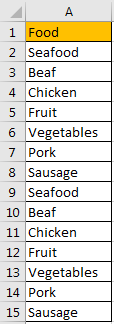Step 1: In any cell except A1:A15, enter the formula =IFERROR(INDEX(\$A\$2:\$A\$15, MATCH(0,COUNTIF(\$C\$1:C1, \$A\$2:\$A\$15), 0)),””).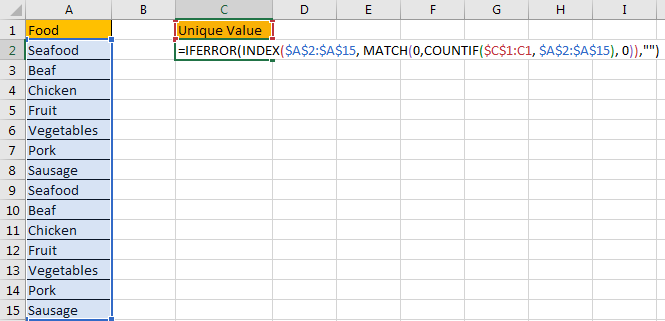Step 2: As it is an array formula, so we need to press Ctrl+Shift+Enter simultaneously to get result.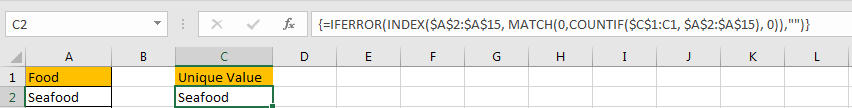We get Seafood in this location.

Step 3: Drag the fill handle down to fill the other cells in C column. Till there is nothing to be shown in cell.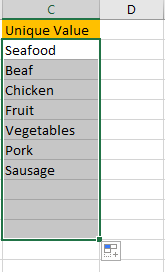So, the list in C column records all unique values from selected range.

Step 4: After above steps, we get all unique values from selected range. Now let’s try to update one value in selected range, and verify if C column can be updated accordingly.

For example, update the second ‘Seafood’ to ‘Orange Juice’.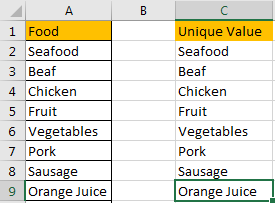Verify that a new value is added in the end of Unique Value list directly. That means above formula works well and applies on C column properly.

## Dynamically Extract Unique Values from A Column List by VBA Code

If you are familiar with coding, you can also through editing VBA code to implement extracting unique values dynamically.

Step 1: On current visible worksheet, right click on sheet name tab to load Sheet management menu. Select View Code, Microsoft Visual Basic for Applications window pops up.

Or you can enter Microsoft Visual Basic for Applications window via Developer->Visual Basic. You can also press Alt + F11 keys simultaneously to open it.

Step 2: In Microsoft Visual Basic for Applications window, click Insert->Module, enter below code in Module1:

```Sub ExtractUniqueValue()

Dim xRng As Range

Dim xRow1 As Long

Dim xRow2 As Long

Dim i As Integer

On Error Resume Next

Set xRng = Application.InputBox("Please select range:", "Extract Unique Value", Selection.Address, , , , , 8)

If xRng Is Nothing Then Exit Sub

On Error Resume Next

xRng.Copy Range("C2")

xRow1 = xRng.Rows.Count + 1

xRow2 = Cells(Rows.Count, "A").End(xlUp).Row

For i = 1 To xLastRow2

If ActiveSheet.Range("C2:C" & xRow2).Cells(i).Value = "" Then

ActiveSheet.Range("C2:C" & xRow2).Cells(i).Delete

End If

Next

End Sub```

Step 3: Save the codes, see screenshot below. And then quit Microsoft Visual Basic for Applications.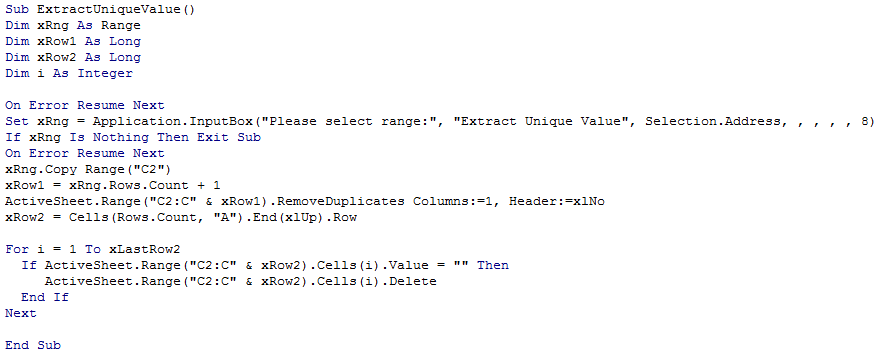Step 4: Click Developer->Macros to run Macro. Select ‘ExtractUniqueValue’ and click Run.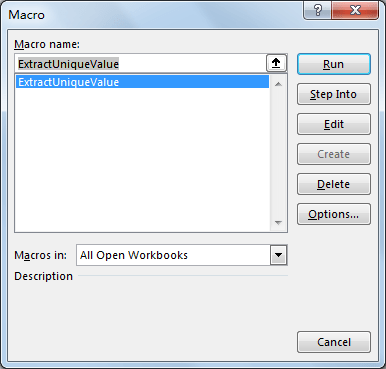Step 5: Extract Unique Value dialog pops up. Enter Select Range \$A\$2:\$A\$15. Click OK. In this step you can select the range you want to extract unique values.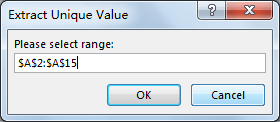Step 6: Click OK and check the result. Verify that unique values are displayed in C column properly. The behavior is as same as the result in method 1 step# 3.

Step 7: If you update the original list, you have to run macro to refresh Unique Value list. This way can also dynamically extract unique values from original list.

### Related Functions

• Excel IFERROR function
The Excel IFERROR function returns an alternate value you specify if a formula results in an error, or returns the result of the formula.The syntax of the IFERROR function is as below:= IFERROR (value, value_if_error)….
• Excel INDEX function
The Excel INDEX function returns a value from a table based on the index (row number and column number)The INDEX function is a build-in function in Microsoft Excel and it is categorized as a Lookup and Reference Function.The syntax of the INDEX function is as below:= INDEX (array, row_num,[column_num])…
• Excel MATCH  function
The Excel MATCH function search a value in an array and returns the position of that item.The MATCH function is a build-in function in Microsoft Excel and it is categorized as a Lookup and Reference Function.The syntax of the MATCH function is as below:= MATCH  (lookup_value, lookup_array, [match_type])….
• Excel COUNTIF function
The Excel COUNTIF function will count the number of cells in a range that meet a given criteria. This function can be used to count the different kinds of cells with number, date, text values, blank, non-blanks, or containing specific characters.etc.= COUNTIF (range, criteria)…

## How to Create Dynamic Drop Down List without Blank in Excel

This post will guide you how to create dynamic drop down list without blank cells in Microsoft Excel.

In Excel, and you can use Data Validation feature to improve the efficiency of data entry in excel, and it also be used to reduce mistake and typing errors. And it is also be used to restrict the user for the type of data that can be entered in the range.

Assuming that you have a list of data in your current worksheet, and you can want to create a dynamic drop down list based on those data without blank cells, and if you use the usual method to create it, and it may be doesn’t work. And you can use an OFFSET formula and count the entries in the column, and calculate the number of rows in the range, and then create a source list without blanks, and use formulas to pull the numbered items into a new column. Just do the following steps:

Step1: select cell A2 next to the original data list B1:B8, and type the following formula in Cell A2, and copy it down to cell A8, and it will number the cells that are not blank.

=IF(B2=””,””,MAX(A\$1:A1)+1)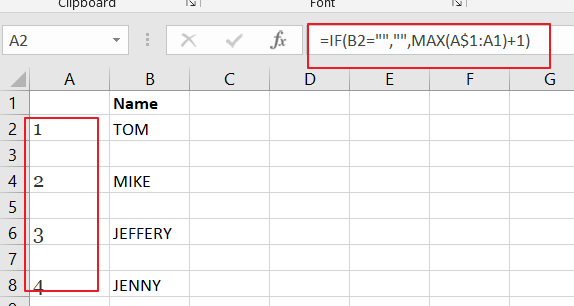Step2: then you can create a new source list column without blanks based on the source data list, just enter the following formula into the cell D2 in a new column, and then copy it down to the cell D8 to create a new data list with all the blanks at the end.

=IFERROR(INDEX(\$B\$2:\$B\$8,MATCH(ROW()-ROW(\$D\$1),\$A\$2:\$A\$8,0)),””)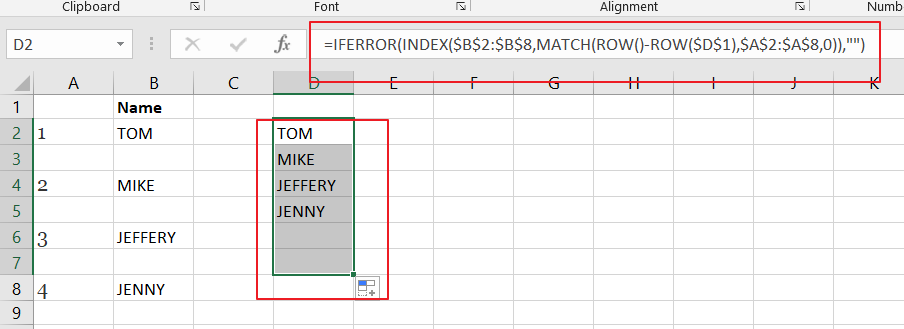Step3: you can create a dynamic drop down list based on the newly source range without blanks. Select a cell that you want to create dynamic drop down list , and go to Data tab, and click Data Validation command under Data Tools group. And the Data Validation dialog will appear.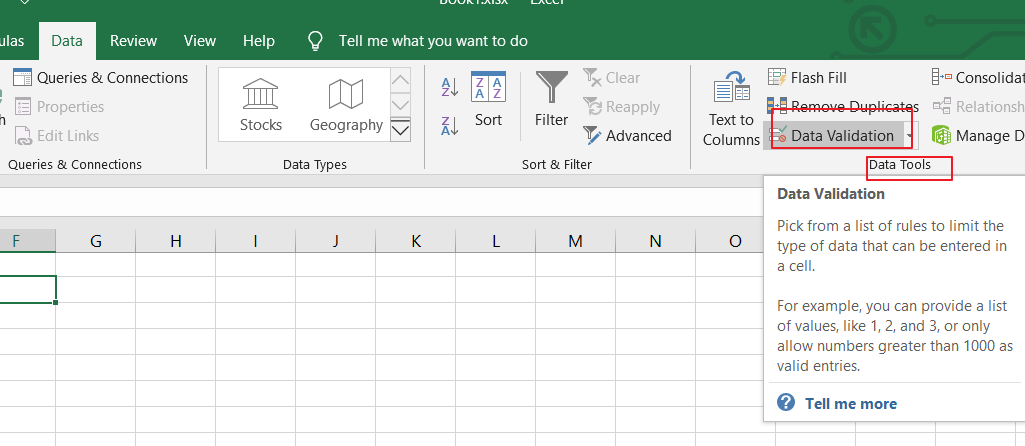Step4: select List value from the Allow drop down list in the Data Validation dialog box, and enter the following formula into the Source text box. And click Ok button.

=OFFSET(\$D\$1,1,0,MAX(\$A:\$A),1)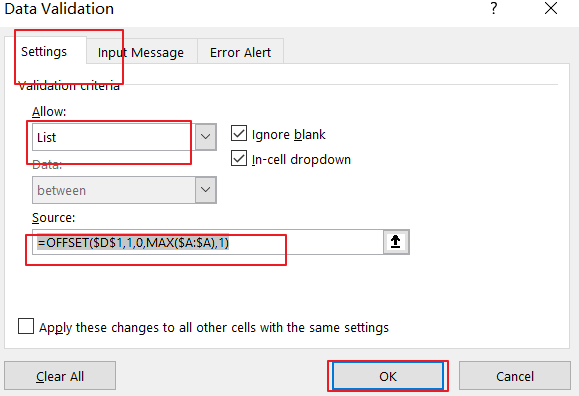Step5: You would see that the dynamic drop down list should be created without blanks in your current worksheet.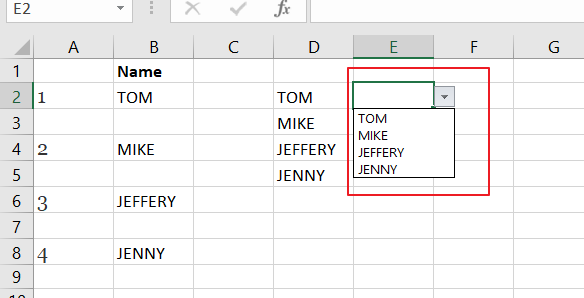### Related Functions

• Excel IF function
The Excel IF function perform a logical test to return one value if the condition is TRUE and return another value if the condition is FALSE. The IF function is a build-in function in Microsoft Excel and it is categorized as a Logical Function.The syntax of the IF function is as below:= IF (condition, [true_value], [false_value])….
• Excel IFERROR function
The Excel IFERROR function returns an alternate value you specify if a formula results in an error, or returns the result of the formula.The syntax of the IFERROR function is as below:= IFERROR (value, value_if_error)….
• Excel INDEX function
The Excel INDEX function returns a value from a table based on the index (row number and column number)The INDEX function is a build-in function in Microsoft Excel and it is categorized as a Lookup and Reference Function.The syntax of the INDEX function is as below:= INDEX (array, row_num,[column_num])…
• Excel MATCH  function
The Excel MATCH function search a value in an array and returns the position of that item.The MATCH function is a build-in function in Microsoft Excel and it is categorized as a Lookup and Reference Function.The syntax of the MATCH function is as below:= MATCH  (lookup_value, lookup_array, [match_type])….
• Excel MAX function
The Excel MAX function returns the largest numeric value from the numbers that you provided. Or returns the largest value in the array.= MAX(num1,[num2,…numn])…
• Excel ROW function
The Excel ROW function returns the row number of a cell reference.The ROW function is a build-in function in Microsoft Excel and it is categorized as a Lookup and Reference Function.The syntax of the ROW function is as below:= ROW ([reference])….

## How to Compare Two Columns for Differences in Excel

This post will guide you how to compare two columns and return differences in Excel. How do I compare two columns to find differences in Excel 2013/2016.

## Compare Two Columns for Differences

Assuming that you have a list of data in range A1:B5, and you want to compare Column A and Column B and return the differences from these two columns. You can use an formula based on the IF function, the ISERROR function and the MATCH function to compare those two columns and find the differences.  like this:

if you want to get the differences between column A and Column B, just use the following formula:

=IF((ISERROR(MATCH(A1,\$B\$1:\$B\$5,0))),A1,””)

type this formula into a blank cell and press Enter key on your keyboard, and then drag the AutoFill Handle down to other cells.  You would see the differences.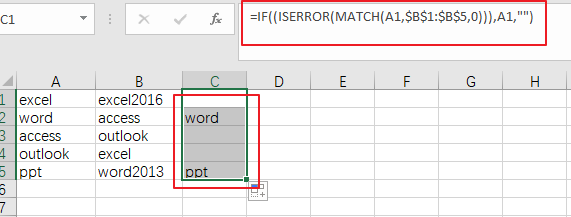### Related Functions

• Excel IF function
The Excel IF function perform a logical test to return one value if the condition is TRUE and return another value if the condition is FALSE. The IF function is a build-in function in Microsoft Excel and it is categorized as a Logical Function.The syntax of the IF function is as below:= IF (condition, [true_value], [false_value])….
• Excel IFERROR function
The Excel IFERROR function returns an alternate value you specify if a formula results in an error, or returns the result of the formula.The syntax of the IFERROR function is as below:= IFERROR (value, value_if_error)….
• Excel MATCH function
The Excel MATCH function search a value in an array and returns the position of that item.The syntax of the MATCH function is as below:= MATCH  (lookup_value, lookup_array, [match_type])….

## How to Vlookup to Return Blank Instead of 0 or NA Error in Excel

This post will guide you how to vlookup and return blank instead of 0 or #N/A error in Excel. How do I vlookup returning blank instead of 0 or #N/A when no data matched in Excel.

## Vlookup to Return Blank Instead of 0 or #N/A

When you use vlookup function to search a value, and it will return the searched value, and if the matched cell is blank, it will return 0. Or if the matched cell is not found, it will return #N/A error.  If you do not want to return blank instead of 0 or #N/A for those two cases. How to use the VLOOKUP function to create a formula in Excel.

Assuming that you have a list of data in Range of Cells A1:B5, and you want to find “excel” text string in range A1:B5, and return the corresponding value in column B.

If you want to return blank instead of 0 or #N/A Error, you can use a formula based on the IFERROR function, the IF function, the LEN function and the VLOOKUP function. Like this:

`=IFERROR(IF(LEN(VLOOKUP("excel",A1:B5,2,0))=0,"",VLOOKUP("excel",A1:B5,2,0)), "")`

Type this formula into a blank cell, and press Enter key in your keyboard.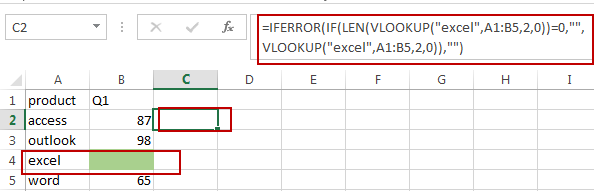Let’s see how this formula works:

The first VLOOKUP formula will lookup value “excel” in range A1:B5, if TRUE, then return the corresponding value in Column B. and then the returned value passed into LEN function as it argument.

The Len function will return the length of the returned value by VLOOKUP Function. If the length is equal to 0, it indicates that the matching value is blank.

The IF formula will check if the length value is 0 or not, if it is equal to 0, then return blank value, otherwise, return the matched value.

The IFERROR function will check if the returned value by IF is #N/A, if true, return blank value.

### Related Functions

• Excel IF function
The Excel IF function perform a logical test to return one value if the condition is TRUE and return another value if the condition is FALSE. The IF function is a build-in function in Microsoft Excel and it is categorized as a Logical Function.The syntax of the IF function is as below:= IF (condition, [true_value], [false_value])….
• Excel VLOOKUP function
The Excel VLOOKUP function lookup a value in the first column of the table and return the value in the same row based on index_num position.The syntax of the VLOOKUP function is as below:= VLOOKUP (lookup_value, table_array, column_index_num,[range_lookup])….
• Excel IFERROR function
The Excel IFERROR function returns an alternate value you specify if a formula results in an error, or returns the result of the formula.The syntax of the IFERROR function is as below:= IFERROR (value, value_if_error)….
• Excel LEN function
The Excel LEN function returns the length of a text string (the number of characters in a text string).The syntax of the LEN function is as below:= LEN(text)…

## VLOOKUP Values from Multiple Worksheets

This post will guide you how to use the VLOOKUP function to find the values from multiple worksheets in Excel. For example, assuming that you have two worksheets, and you want to search the data across those two worksheet in your current worksheet (sheet3). How to do it. How to use the VLOOKUP function to search value across two or more worksheets in Excel.

You can create a new formula based on the VLOOKUP function and the IFERROR function as follows:

`=IFERROR(VLOOKUP(\$A1,Sheet1!\$A\$1:\$B\$4,2,FALSE), VLOOKUP(\$A1,Sheet2!\$A\$1:\$B\$4,2,FALSE))`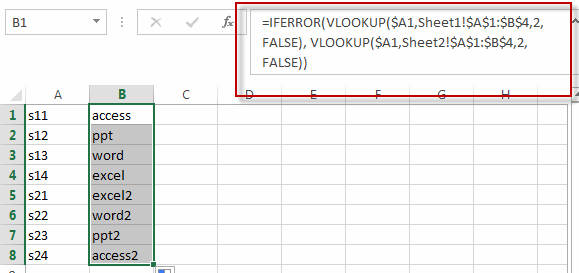Let’s see how this formula works:

=VLOOKUP(\$A1,Sheet1!\$A\$1:\$B\$4,2,FALSE)

The VLOOKUP formula will search the value of Cell A1(sheet3) in the first column in the range A1:B4 of worksheet sheet1, then return an exact match or it will return an error value #N/A.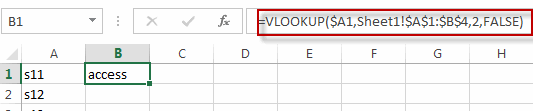=VLOOKUP(\$A1,Sheet2!\$A\$1:\$B\$4,2,FALSE)

This formula will search the value of Cell A1(sheet3) in the first column in the range A1:B4 of another worksheet sheet2. Then it will return an exact match from column 2 that is in the same row. Or it will return the error value #N/A.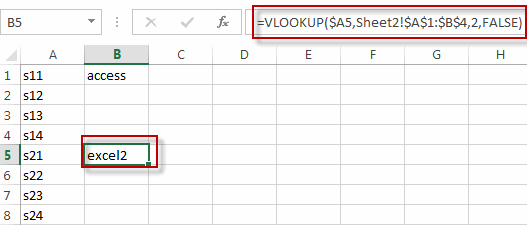=IFERROR()

This formula will check if the first VLOOKUP return an error value #N/A, if TRUE, then return the value of the second VLOOKUP function. If FALSE, then return the value of the first VLOOKUP function.

If you want to VLOOKUP values from three or more worksheet, then you can write down the following formula:

`=IFERROR(VLOOKUP(\$A1,Sheet1!\$A\$1:\$B\$4,2,FALSE), IFERROR(VLOOKUP(\$A1,Sheet2!\$A\$1:\$B\$4,2,FALSE), VLOOKUP(\$A1,Sheet3!\$A\$1:\$B\$4,2,FALSE)))`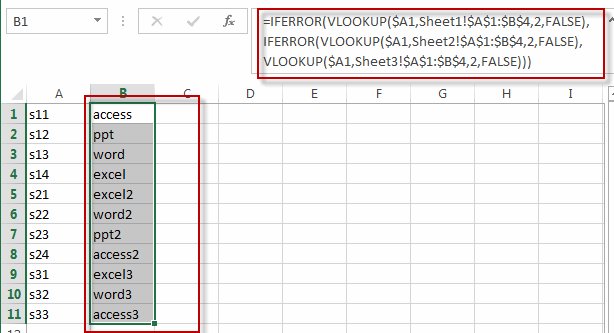### Related Functions

• Excel VLOOKUP function
The Excel VLOOKUP function lookup a value in the first column of the table and return the value in the same row based on index_num position.The syntax of the VLOOKUP function is as below:= VLOOKUP (lookup_value, table_array, column_index_num,[range_lookup])….
• Excel IFERROR function
The Excel IFERROR function returns an alternate value you specify if a formula results in an error, or returns the result of the formula.The syntax of the IFERROR function is as below:= IFERROR (value, value_if_error)….

## How to Get the List of File Names From a Folder

This post will teach you how to get the list of file names in a given directory to a worksheet in Excel. You will learn that how to use Excel to view Files and Folders in your worksheet by get the list of file names with different ways, such as: Excel VBA Macro, or FILES function.

If you want to get the list of file names from a folder that contain hundreds of files in it. You may be think the simplest way is that selecting the file and copying its name, then paste the file name in a cell in your worksheet, then repeat the above steps. If you follow this way to do it, you will take a long time to complete it. So it is a simple way, but it is also a huge waste of time. Do we have some quick way to achieve the same result? Of course yes, the below will talk two ways to copy the list of file names from a folder into worksheet quickly.

## Method 1: Using FILES function to get list of file names

You can use the FILES function to get the list of file names from a directory, and this function is only works in name Defined Names. And then you can use a combination of the IFERROR function, the INDEX function and the Row function to create an excel formula. Let’s see the below steps:

1# Assuming that you want to get the list of file names from a directory, such as: C:\Users\devops\Tracing\WPPMedia\*, and enter this directory path into Cell B1.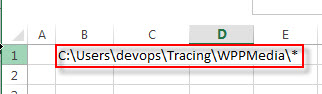2# On the FORMULAS Tab, click Define Name command under Defined Names group, then select Define Names… from the drop-down menu list.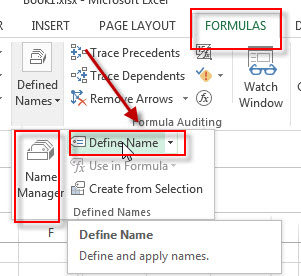3# the New Name window will appear. Set a name in the Name box, such as: FileNameList, type the following formula in the Refers to box.

=FILES(Sheet7!\$B\$1)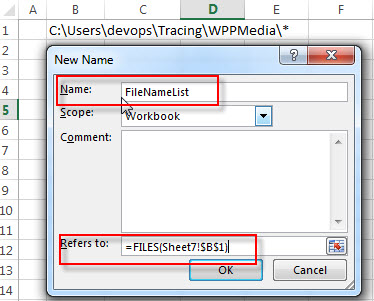4# enter the following formula in the Cell B3, then drag the AutoFill Handle down to others cells to apply this formula.

`=IFERROR(INDEX(FileNameList,ROW()-2)," ")`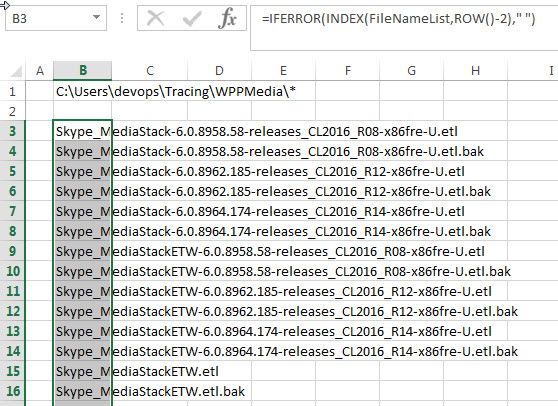You will see that all file names from the specified folder are listed in your worksheet.

## Method 2: Using File browser and web browser to get list of file names

If you want to use this method to get the list of file name from a directory, you need to install one web browser, such as: Firefox, Chrome or IE. Then do it follow steps:

1# Move to the destination folder or directory on the Windows File Explorer, then copy the path of that directory. Such as: C:\Users\devops\Tracing\WPPMedia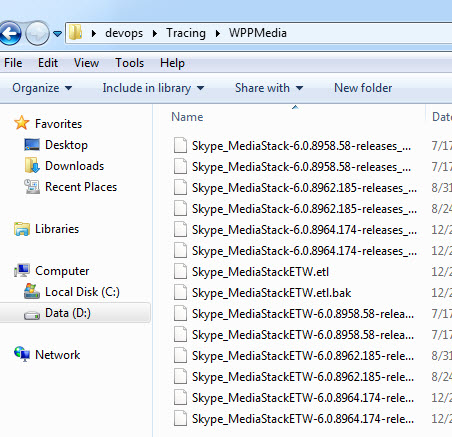2# open any web browser installed in your computer and paste the copied path in the address bar, then press Enter key.

3# you will see that it will add prefix file:/// at the beginning of the path in the address bar. And the file name list will be shown in the web page.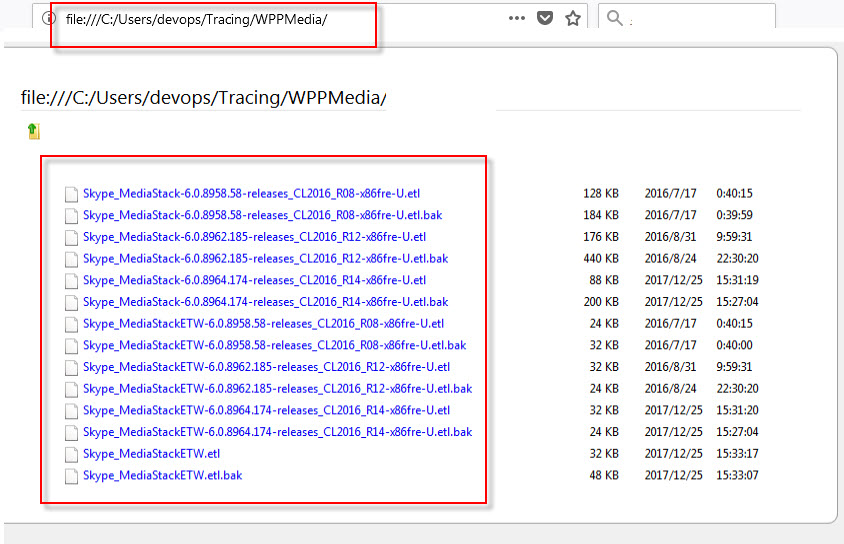4# press Ctrl + A shortcut to select all of file names in the web page and then press Ctrl +C to copy the selected content.

5# open your worksheet, then press Ctrl + V to paste all file names into your worksheet.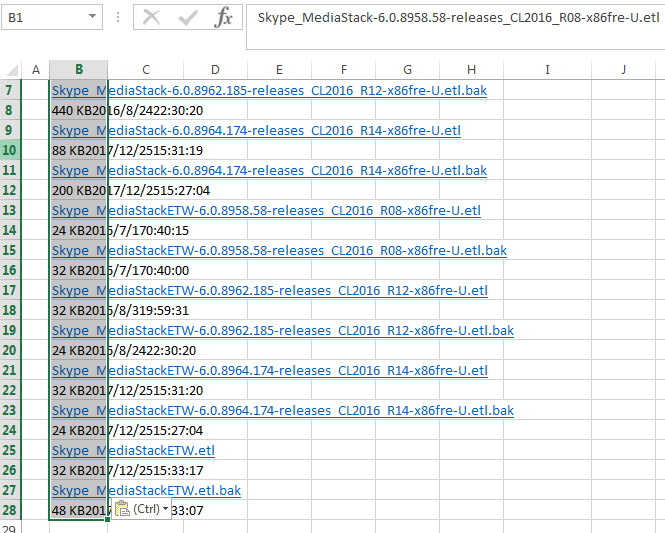Or you can save the web page in the Step 3 as the offline copy. Just press Ctrl + S or right-click on the web page, and then select Save Page As to save that web page.

Open the saved web page from the web browser, and copy its web address. Then click From WEB command under Get External Data group on the DATA tab in your worksheet.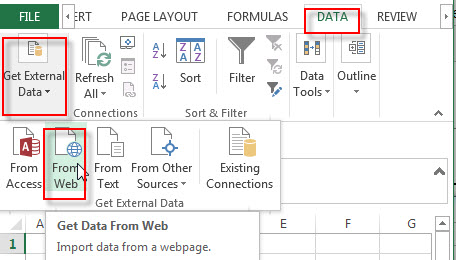Paste the web address in Address box, then click Go button. Then click Import button. You will see that all files and folders details are imported to your worksheet.

## Method 3: Using Excel VBA macro to get list of file names

You can write an excel VBA macro code to get the list of file names from a specified directory,

1# click on “Visual Basic” command under DEVELOPER Tab.2# then the “Visual Basic Editor” window will appear.

3# click “Insert” ->”Module” to create a new module4# paste the below VBA code into the code window. Then clicking “Save” button.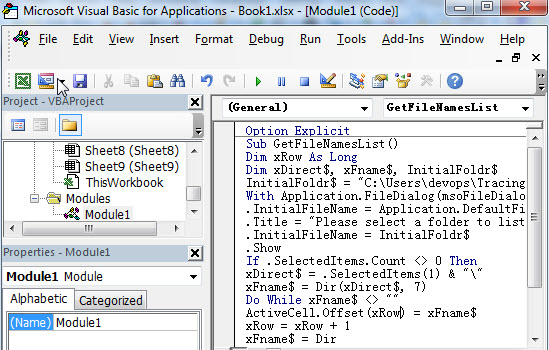```Option Explicit
Sub GetFileNames()
Dim xRow As Long
Dim xDirect\$, xFname\$, InitialFoldr\$
InitialFoldr\$ = "C:\"
With Application.FileDialog(msoFileDialogFolderPicker)
.InitialFileName = Application.DefaultFilePath & "\"
.Title = "Please select a folder to list Files from"
.InitialFileName = InitialFoldr\$
.Show
If .SelectedItems.Count <> 0 Then
xDirect\$ = .SelectedItems(1) & "\"
xFname\$ = Dir(xDirect\$, 7)
Do While xFname\$ <> ""
ActiveCell.Offset(xRow) = xFname\$
xRow = xRow + 1
xFname\$ = Dir
Loop
End If
End With
End Sub```

5# back to the current worksheet, then run the above excel macro.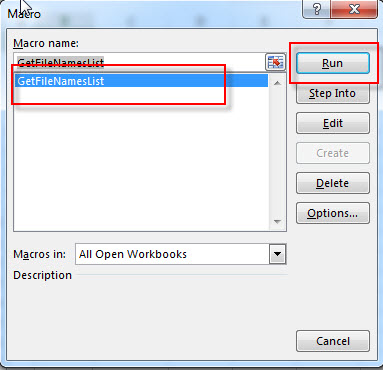6# select a folder that you want to get all names, then click OK button.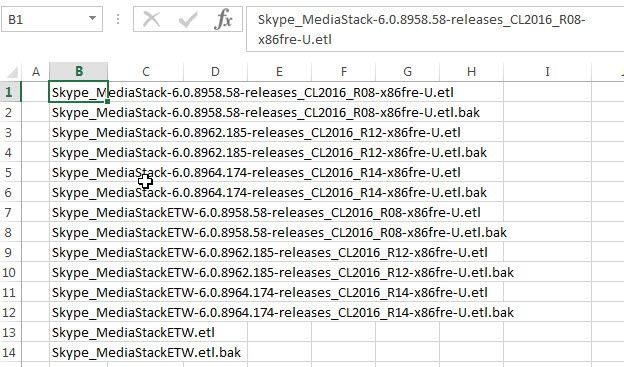### Related Functions

• Excel IFERROR function
The Excel IFERROR function returns an alternate value you specify if a formula results in an error, or returns the result of the formula.The syntax of the IFERROR function is as below:= IFERROR (value, value_if_error)…
• Excel INDEX function
The Excel INDEX function returns a value from a table based on the index (row number and column number)The INDEX function is a build-in function in Microsoft Excel and it is categorized as a Lookup and Reference Function.The syntax of the INDEX function is as below:= INDEX (array, row_num,[column_num])…
• Excel ROW function
The Excel ROW function returns the row number of a cell reference.The ROW function is a build-in function in Microsoft Excel and it is categorized as a Lookup and Reference Function.The syntax of the ROW function is as below:= ROW ([reference])….